# Duncan Lardeau Juvenile Rainbow Trout Abundance 2019b

The suggested citation for this analytic appendix is:

Thorley, J.L. and Amies-Galonski, E. (2019) Duncan Lardeau Juvenile Rainbow Trout Abundance 2019b. A Poisson Consulting Analysis Appendix. URL: https://www.poissonconsulting.ca/f/1245769249.

## Background

Rainbow Trout rear in the Lardeau and Lower Duncan rivers. Since 2006 (with the exception of 2015) annual spring snorkel surveys have been conducted to estimate the abundance and distribution of age-1 Rainbow Trout. From 2006 to 2010 the surveys were conducted at fixed index sites. Since 2011 fish observations have been mapped to the river based on their spatial coordinates as recorded by GPS.

The primary aims of the current analyses were to:

• Estimate the abundance of age-1 and age-2 fish by year.
• Estimate the egg deposition.
• Estimate the stock-recruitment relationship between the egg deposition and the abundance of age-1 recruits the following spring.
• Estimate the survival from age-1 to age-2.
• Estimate the expected number of spawners without in river and/or in lake variation.

## Methods

### Data Preparation

The snorkel count data were provided by the Ministry of Forests, Lands and Natural Resource Operations (MFLNRO). The data were manipulated using R version 3.6.1 (R Core Team 2019).

Key assumptions of the data preparation included:

• Counts in visibilities less than 3 m where unreliable.
• Age-1 individuals were those with a fork length $$\leq$$ 100 mm while age-2 individual were those with a fork length $$>$$ 100 and $$\leq$$ 160 mm.

### Statistical Analysis

Model parameters were estimated using Maximum-Likelihood (ML) and Bayesian methods. The Maximum-Likelihood Estimates (MLE) were obtained using TMB (Kristensen et al. 2016) while the Bayesian estimates were produced using JAGS (Plummer 2015) and STAN (Carpenter et al. 2017). For additional information on ML and Bayesian estimation the reader is referred to Millar (2011) and McElreath (2016), respectively. ML models have the advantage of being relatively quick to fit while Bayesian models capture all the uncertainty and allow intuitive probabilistic statements.

Unless stated otherwise, the Bayesian analyses used weakly informative normal and half-normal prior distributions (Gelman, Simpson, and Betancourt 2017). The posterior distributions were estimated from 1500 Markov Chain Monte Carlo (MCMC) samples thinned from the second halves of 3 chains (Kery and Schaub 2011, 38–40). Model convergence was confirmed by ensuring that the potential scale reduction factor $$\hat{R} \leq 1.05$$ (Kery and Schaub 2011, 40) and the effective sample size (Brooks et al. 2011) $$\textrm{ESS} \geq 150$$ for each of the monitored parameters (Kery and Schaub 2011, 61).

The parameters are summarised in terms of the point estimate, standard deviation (sd), the z-score, lower and upper 95% confidence/credible limits (CLs) and the p-value (Kery and Schaub 2011, 37, 42). For ML models, the point estimate is the MLE, the standard deviation is the standard error, the z-score is $$\mathrm{MLE}/\mathrm{sd}$$ and the 95% CLs are the $$\mathrm{MLE} \pm 1.96 \cdot \mathrm{sd}$$. For Bayesian models, the estimate is the median (50th percentile) of the MCMC samples, the z-score is $$\mathrm{mean}/\mathrm{sd}$$ and the 95% CLs are the 2.5th and 97.5th percentiles. A p-value of 0.05 indicates that the lower or upper 95% CL is 0.

Model averaging and selection was implemented using an information theoretic approach (Burnham and Anderson 2002). ML models were compared using the marginal Akaike’s Information Criterion corrected for small sample size (AICc, Burnham and Anderson 2002) while Bayesian models were compared using the Watanabe-Akaike Information Criterion (WAIC, Watanabe 2010). The support for fixed terms in models with identical random (Kery and Schaub 2011, 75) effects was assessed using the Akaike’s weights ($$w_i$$) which were also used for model averaging (Burnham and Anderson 2002). The top ML model for each analysis was refitted as a Bayesian model. Where relevant, model adequacy was confirmed by examination of residual plots for the full model(s).

The results are displayed graphically by plotting the modeled relationships between particular variables and the response(s) with the remaining variables held constant. In general, continuous and discrete fixed variables are held constant at their mean and first level values, respectively, while random variables are held constant at their typical values (expected values of the underlying hyperdistributions) (Kery and Schaub 2011, 77–82). When informative the influence of particular variables is expressed in terms of the effect size (i.e., percent change in the response variable) with 95% confidence/credible intervals (CIs, Bradford, Korman, and Higgins 2005).

The analyses were implemented using R version 3.6.1 (R Core Team 2019) and the mbr family of packages.

### Model Descriptions

#### Length Correction

The annual bias (inaccuracy) and error (imprecision) in observer’s fish length estimates when spotlighting (standing) and snorkeling were quantified from the divergence of their length distribution from the length distribution for all observers (including measured fish) in that year. More specifically, the length correction that minimised the Jensen-Shannon divergence (Lin 1991) between the two distributions provided a measure of the inaccuracy while the minimum divergence (the Jensen-Shannon divergence was calculated with log to base 2 which means it lies between 0 and 1) provided a measure of the imprecision.

#### Abundance

The abundance was estimated from the count data using an overdispersed Poisson model (Kery and Schaub 2011, 55–56). The annual abundance estimates represent the total number of fish in the study area.

Key assumptions of the abundance model include:

• The lineal fish density varies with year, river kilometer as a polynomial, and randomly with site.
• The observer efficiency at marking sites varies by study design (GPS versus Index).
• The observer efficiency also varies by visit type (marking versus count) within study design and randomly by snorkeller.
• The expected count at a site is the expected lineal density multiplied by the site length, the observer efficiency and the proportion of the site surveyed.
• The residual variation in the actual count is gamma-Poisson distributed.

#### Condition

The condition of fish with a fork length $$\geq$$ 500 mm was estimated via an analysis of mass-length relations (He et al. 2008).

More specifically the model was based on the allometric relationship

$W = \alpha_c L^{\beta_c}$

where $$W$$ is the weight (mass), $$\alpha_c$$ is the coefficent, $$\beta_c$$ is the exponent and $$L$$ is the length.

To improve chain mixing the relation was log-transformed, i.e.,

$\log(W) = \log(\alpha_c) + \beta_c \log(L).$

Key assumptions of the condition model include:

• $$\alpha_c$$ can vary randomly by year.
• The residual variation in weight is log-normally distributed.

#### Fecundity

The fecundity of females with a fork length $$\geq$$ 500 mm was estimated via an analysis of fecundity-mass relations.

More specifically the model was based on the allometric relationship

$F = \alpha_f W^{\beta_f}$

where $$F$$ is the fecundity, $$\alpha_f$$ is the coefficent, $$\beta_f$$ is the exponent and $$W$$ is the weight.

To improve chain mixing the relation was log-transformed.

Key assumptions of the fecundity model include:

• The residual variation in fecundity is log-normally distributed.

#### Spawner Size

The average length of the spawners in each year (for years for which it was unavailable) was estimated from the mean weight of Rainbow Trout in the Kootenay Lake Rainbow Trout Mailout Survey (KLRT) using a linear regression. This approach was suggested by Rob Bison.

#### Egg Deposition

The egg deposition in each year was estimated by

1. converting the average length of spawners to the average weight using the condition relationship for a typical year
2. adjusting the average weight by the annual condition effect (interpolating where unavailable)
3. converting the average weight to the average fecundity using the fecundity relationship
4. multiplying the average fecundity by the AUC based estimate of the number of females (assuming a sex ratio of 1:1)

#### Stock-Recruitment

The relationship between the number of eggs ($$E$$) and the abundance of age-1 individuals the following spring ($$R$$) was estimated using a Beverton-Holt stock-recruitment model (Walters and Martell 2004):

$R = \frac{\alpha_s \cdot E}{1 + \beta_s \cdot E} \quad,$

where $$\alpha_s$$ is the maximum number of recruits per egg (egg survival), and $$\beta_s$$ is the density dependence.

Key assumptions of the stock-recruitment model include:

• The residual variation in the number of recruits is log-normally distributed with the standard deviation scaling with the uncertainty in the number of recruits.

The age-1 carrying capacity ($$K$$) is given by:

$K = \frac{\alpha_s}{\beta_s} \quad.$ and the $$E_{K/2}$$ Limit Reference Point (Mace 1994, E_{0.5 R_{max}}), which corresponds to the stock (number of eggs) that produce 50% of the maximum recruitment ($$K$$), by $E_{K/2} = \frac{1}{\beta_s}$

The LRP was also converted into a number of spawners in a typical year (assuming 6,000 eggs per spawner and a sex ratio of 1:1).

#### Age-1 to Age-2 Survival

The relationship between the number of age-1 individuals and the number of age-2 individuals the following year was estimated using a linear regression through the origin where the slope was constrained to lie between 0 and 1 by a logistic transformation.

Key assumptions of the survival rate model include:

• The residual variation in the number of age-2 individuals is log-normally distributed.

#### Reproductive Rate

The maximum reproductive rate (the number of spawners per spawner at low density) not accounting for fishing mortality was calculated by multiplying $$\beta_s$$ (number of recruits per egg at low density) from the stock-recruitment relationship by the inlake survival by the average number of eggs per spawner in a typical year (assumed to be 3,000 based on 6,000 eggs per spawner and a sex ratio of 1:1). The inlake survival from age-1 to spawning was calculated by dividing the subsequent number of spawners by the number of recruits assuming that equal numbers of fish spawn at age 5, 6 and 7.

#### Expected Spawners

The expected spawners without in river and/or in lake variation was calculated from the stock-recruitment relationship and assuming in age-1 to spawner survival of 0.66%.

## Results

### Model Templates

#### Abundance

  data {

int<lower=0> nMarked;
int<lower=0> Marked[nMarked];
int<lower=0> Resighted[nMarked];
int<lower=0> IndexMarked[nMarked];

int<lower=0> nObs;
int Marking[nObs];
int Index[nObs];
int<lower=0> nSwimmer;
int<lower=0> Swimmer[nObs];
int<lower=0> nYear;
int<lower=0> Year[nObs];
real Rkm[nObs];
int<lower=0> nSite;
int<lower=0> Site[nObs];

real SiteLength[nObs];
real SurveyProportion[nObs];

int Count[nObs];
}
parameters {
real bEfficiency;
real bEfficiencyIndex;

real bDensity;
real<lower=0> sDensityYear;
vector[nYear] bDensityYear;

vector bDensityRkm;
real<lower=0> sDensitySite;
vector[nSite] bDensitySite;

real bEfficiencyMarking;
real bEfficiencyMarkingIndex;

real<lower=0,upper=5> sEfficiencySwimmer;
vector[nSwimmer] bEfficiencySwimmer;

real<lower=0> sDispersion;
}
model {

vector[nObs] eDensity;
vector[nObs] eEfficiency;
vector[nObs] eAbundance;
vector[nObs] eCount;

sDispersion ~ gamma(0.01, 0.01);

bDensity ~ normal(0, 2);
bDensityRkm ~ normal(0, 2);
sDensitySite ~ uniform(0, 5);
bDensitySite ~ normal(0, sDensitySite);
sDensityYear ~ uniform(0, 5);
bDensityYear ~ normal(0, sDensityYear);

bEfficiency ~ normal(0, 5);
bEfficiencyIndex ~ normal(0, 5);
bEfficiencyMarking ~ normal(0, 5);
bEfficiencyMarkingIndex ~ normal(0, 5);
sEfficiencySwimmer ~ uniform(0, 5);
bEfficiencySwimmer ~ normal(0, sEfficiencySwimmer);

for (i in 1:nMarked) {
target += binomial_lpmf(Resighted[i] | Marked[i],
inv_logit(
bEfficiency +
bEfficiencyIndex * IndexMarked[i] +
bEfficiencyMarking +
bEfficiencyMarkingIndex * IndexMarked[i]
));
}

for (i in 1:nObs) {
eDensity[i] = exp(bDensity +
bDensityRkm * Rkm[i] +
bDensityRkm * pow(Rkm[i], 2.0) +
bDensityRkm * pow(Rkm[i], 3.0) +
bDensityRkm * pow(Rkm[i], 4.0) +
bDensitySite[Site[i]] +
bDensityYear[Year[i]]);

eEfficiency[i] = inv_logit(
bEfficiency +
bEfficiencyIndex * Index[i] +
bEfficiencyMarking * Marking[i] +
bEfficiencyMarkingIndex * Index[i] * Marking[i] +
bEfficiencySwimmer[Swimmer[i]]);

eAbundance[i] = eDensity[i] * SiteLength[i];

eCount[i] = eAbundance[i] * eEfficiency[i] * SurveyProportion[i];
}

target += neg_binomial_2_lpmf(Count | eCount, sDispersion);
}

Block 1. Abundance model description.

#### Condition

 data {
int nYear;
int nObs;

vector[nObs] Length;
vector[nObs] Weight;
int Year[nObs];

parameters {
real bWeight;
real bWeightLength;
real sWeightYear;

vector[nYear] bWeightYear;
real sWeight;

model {

vector[nObs] eWeight;

bWeight ~ normal(-10, 5);
bWeightLength ~ normal(3, 2);

sWeightYear ~ normal(-2, 5);

for (i in 1:nYear) {
bWeightYear[i] ~ normal(0, exp(sWeightYear));
}

sWeight ~ normal(-2, 5);
for(i in 1:nObs) {
eWeight[i] = bWeight + bWeightLength * log(Length[i]) + bWeightYear[Year[i]];
Weight[i] ~ lognormal(eWeight[i], exp(sWeight));
}
..

Block 2.

#### Fecundity

 data {
int nObs;

vector[nObs] Weight;
vector[nObs] Fecundity;

parameters {
real bFecundity;
real bFecundityWeight;
real sFecundity;

model {

vector[nObs] eFecundity;

bFecundity ~ uniform(0, 5);
bFecundityWeight ~ uniform(0, 2);

sFecundity ~ uniform(0, 1);
for(i in 1:nObs) {
eFecundity[i] = log(bFecundity) + bFecundityWeight * log(Weight[i]);
Fecundity[i] ~ lognormal(eFecundity[i], sFecundity);
}
..

Block 3.

#### Stock-Recruitment

model {
a ~ dunif(0, 1)
b ~ dunif(0, 0.1)
sScaling ~ dunif(0, 5)

eRecruits <- a * Stock / (1 + Stock * b)

for(i in 1:nObs) {
esRecruits[i] <- SDLogRecruits[i] * sScaling
Recruits[i] ~ dlnorm(log(eRecruits[i]), esRecruits[i]^-2)
}
..

Block 4. Stock-Recruitment model description.

#### Age-1 to Age-2 Survival

model {
bSurvival ~ dnorm(0, 2^-2)
sRecruits ~ dnorm(0, 2^-2) T(0,)

for(i in 1:nObs) {
logit(eSurvival[i]) <- bSurvival
eRecruits[i] <- Stock[i] * eSurvival[i]
Recruits[i] ~ dlnorm(log(eRecruits[i]), sRecruits^-2)
}
..

Block 5. In-river survival model description.

### Tables

#### Abundance

Table 1. Parameter descriptions.

Parameter Description
bDensity Intercept for log(eDensity)
bDensityRkm[i] ith-order polynomial coefficients of effect of river kilometer on bDensity
bDensitySite[i] Effect of ith Site on bDensity
bDensityYear[i] Effect of ith Year on bDensity
bEfficiency Intercept of logit(eEfficiency)
bEfficiencyIndex Effect of Index on bEfficiency
bEfficiencyMarking Effect of Marking on bEfficiency
bEfficiencyMarkingIndex Effect of Marking and Index on bEfficiency
bEfficiencySwimmer[i] Effect of ith Swimmer on bEfficiency
eAbundance[i] Expected abundance of fish at site of ith visit
eCount[i] Expected total number of fish at site of ith visit
eDensity[i] Expected lineal density of fish at site of ith visit
eEfficiency[i] Expected observer efficiency on ith visit
Index Whether the ith visit was to an index site
Marking[i] Whether the ith visit was to a site with marked fish
Rkm[i] River kilometer of ith visit
sDensitySite SD of bDensitySite
sDispersion Overdispersion of Count[i]
sEfficiencySwimmer SD of bEfficiencySwimmer
Site[i] Site of ith visit
SiteLength[i] Length of site of ith visit
SurveyProportion[i] Proportion of site surveyed on ith visit
Swimmer[i] Snorkeler on ith site visit
Year[i] Year of ith site visit
##### Age-1

Table 2. Model coefficients.

term estimate sd zscore lower upper pvalue
bDensity -1.1096785 0.2736116 -4.036715 -1.6271315 -0.5654508 0.0007
bDensityRkm -0.3670116 0.0770758 -4.715686 -0.5100923 -0.2108058 0.0007
bDensityRkm 0.5743347 0.1043470 5.511545 0.3730466 0.7821185 0.0007
bDensityRkm 0.0460793 0.0365443 1.227622 -0.0290040 0.1135785 0.2293
bDensityRkm -0.2837399 0.0364228 -7.793144 -0.3576818 -0.2134917 0.0007
bEfficiency -1.7369048 0.1540789 -11.254875 -2.0262030 -1.4315869 0.0007
bEfficiencyIndex 0.4557351 0.3498358 1.291327 -0.2143244 1.1596908 0.1933
bEfficiencyMarking 0.5708175 0.1198294 4.776444 0.3290894 0.8066431 0.0007
bEfficiencyMarkingIndex 0.9345531 0.3639227 2.555295 0.2008100 1.6203212 0.0160
sDensitySite 0.6665079 0.0380945 17.513929 0.5959208 0.7448091 0.0007
sDensityYear 0.6493044 0.1752962 3.889189 0.4376515 1.0988435 0.0007
sDispersion 1.3034932 0.0715736 18.248749 1.1718438 1.4532615 0.0007
sEfficiencySwimmer 0.5060444 0.1647808 3.235177 0.2931725 0.9134313 0.0007

Table 3. Model summary.

n K nchains niters nthin ess rhat converged
3251 13 3 500 5 554 1.007 TRUE
##### Age-2

Table 4. Model coefficients.

term estimate sd zscore lower upper pvalue
bDensity -2.1876224 0.2660304 -8.217062 -2.7275112 -1.6832519 0.0007
bDensityRkm -0.1750326 0.0959308 -1.865767 -0.3730968 0.0037882 0.0547
bDensityRkm 0.1693276 0.1305371 1.319051 -0.0870960 0.4238292 0.1800
bDensityRkm 0.0831081 0.0457049 1.833735 -0.0053836 0.1789322 0.0600
bDensityRkm -0.1457071 0.0460659 -3.157077 -0.2328368 -0.0528071 0.0013
bEfficiency -1.8599809 0.2283402 -8.125957 -2.3055024 -1.3902680 0.0007
bEfficiencyIndex 0.4863877 0.3641201 1.356168 -0.1863123 1.2268107 0.1720
bEfficiencyMarking 0.6588288 0.1754703 3.751294 0.3111354 1.0072532 0.0007
bEfficiencyMarkingIndex 2.7874674 0.7233576 3.922076 1.6083104 4.4128190 0.0007
sDensitySite 0.8010971 0.0476260 16.820048 0.7113288 0.8933715 0.0007
sDensityYear 0.5464345 0.1497104 3.847011 0.3624064 0.9532056 0.0007
sDispersion 1.1758854 0.0964979 12.257469 1.0038309 1.3783404 0.0007
sEfficiencySwimmer 0.4017144 0.1270127 3.340744 0.2470735 0.7329242 0.0007

Table 5. Model summary.

n K nchains niters nthin ess rhat converged
3251 13 3 500 5 732 1.006 TRUE

#### Condition

Table 6. Parameter descriptions.

Parameter Description
bWeight Intercept of log(eWeight)
bWeightLength Intercept of effect of log(Length) on bWeight
bWeightYear[i] Effect of ith Year on bWeight
eWeight[i] Expected Weight of ith fish
Length[i] Fork length of ith fish
sWeight Log standard deviation of residual variation in log(Weight)
sWeightYear Log standard deviation of bWeightYear
Weight[i] Recorded weight of ith fish
Year[i] Year ith fish was captured

Table 7. Model coefficients.

term estimate sd zscore lower upper pvalue
bWeight -12.648820 0.2207657 -57.29181 -13.069455 -12.214400 7e-04
bWeightLength 3.203589 0.0329678 97.17575 3.140353 3.266625 7e-04
sWeight -1.905122 0.0205499 -92.70511 -1.944395 -1.864689 7e-04
sWeightYear -2.126987 0.1652127 -12.83389 -2.422576 -1.773743 7e-04

Table 8. Model summary.

n K nchains niters nthin ess rhat converged
1113 4 3 500 2 300 1.014 TRUE

#### Fecundity

Table 9. Parameter descriptions.

Parameter Description
bFecundity Intercept of eFecundity
bFecundityWeight Effect of log(Weight) on log(bFecundity)
eFecundity[i] Expected Fecundity of ith fish
Fecundity[i] Fecundity of ith fish (eggs)
sFecundity SD of residual variation in log(Fecundity)
Weight[i] Weight of ith fish (mm)

Table 10. Model coefficients.

term estimate sd zscore lower upper pvalue
bFecundity 3.8348752 0.9450499 3.883479 1.6222147 4.9332675 7e-04
bFecundityWeight 0.8616885 0.0352672 24.719821 0.8323165 0.9595364 7e-04
sFecundity 0.1261791 0.0209182 6.170162 0.0948517 0.1776660 7e-04

Table 11. Model summary.

n K nchains niters nthin ess rhat converged
22 3 3 500 2 262 1.012 TRUE

#### Stock-Recruitment

Table 12. Parameter descriptions.

Parameter Description
a Recruits per Stock at low density
b Density-dependence
eRecruits[i] Expected number of recruits from ith spawn year
esRecruits[i] Expected SD of residual variation in Recruits
Recruits[i] Number of recruits from ith spawn year
SDLogRecruits[i] Standard deviation of uncertainty in log(Recruits[i])
sScaling Scaling term for SD of residual variation in log(eRecruits)
Stock[i] Number of egg in ith spawn year
##### Age-1

Table 13. Model coefficients.

term estimate sd zscore lower upper pvalue
a 0.5482213 0.2042660 2.828530 0.2451160 0.9632443 7e-04
b 0.0000051 0.0000026 2.125866 0.0000018 0.0000114 7e-04
sScaling 2.2091208 0.5182921 4.446719 1.5198236 3.5136412 7e-04

Table 14. Model summary.

n K nchains niters nthin ess rhat converged
13 3 3 500 100 1371 1.001 TRUE

Table 15. Estimated carry capacity (with 95% CRIs).

estimate lower upper
108000 74100 162000

Table 16. Estimated reference points (with 80% CRIs).

Metric estimate lower upper
eggs 195000 107000.00000 387000
spawners 65 35.66667 129

#### Age-1 to Age-2 Survival

Table 17. Parameter descriptions.

Parameter Description
bSurvival logit(eSurvival)
eSurvival[i] Expected annual survival for ith spawn year
Recruits[i] Number of age-2 juveniles from ith spawn year
sRecruits SD of residual variation in Recruits
Stock[i] Number of age-1 juveniles from ith spawn year
##### Age-2

Table 18. Model coefficients.

term estimate sd zscore lower upper pvalue
bSurvival -1.0184133 0.1690095 -6.025869 -1.339868 -0.6784918 7e-04
sRecruits 0.3830659 0.1061674 3.810358 0.258139 0.6789211 7e-04

Table 19. Model summary.

n K nchains niters nthin ess rhat converged
11 2 3 500 1 590 1.009 TRUE

### Figures

#### Length Correction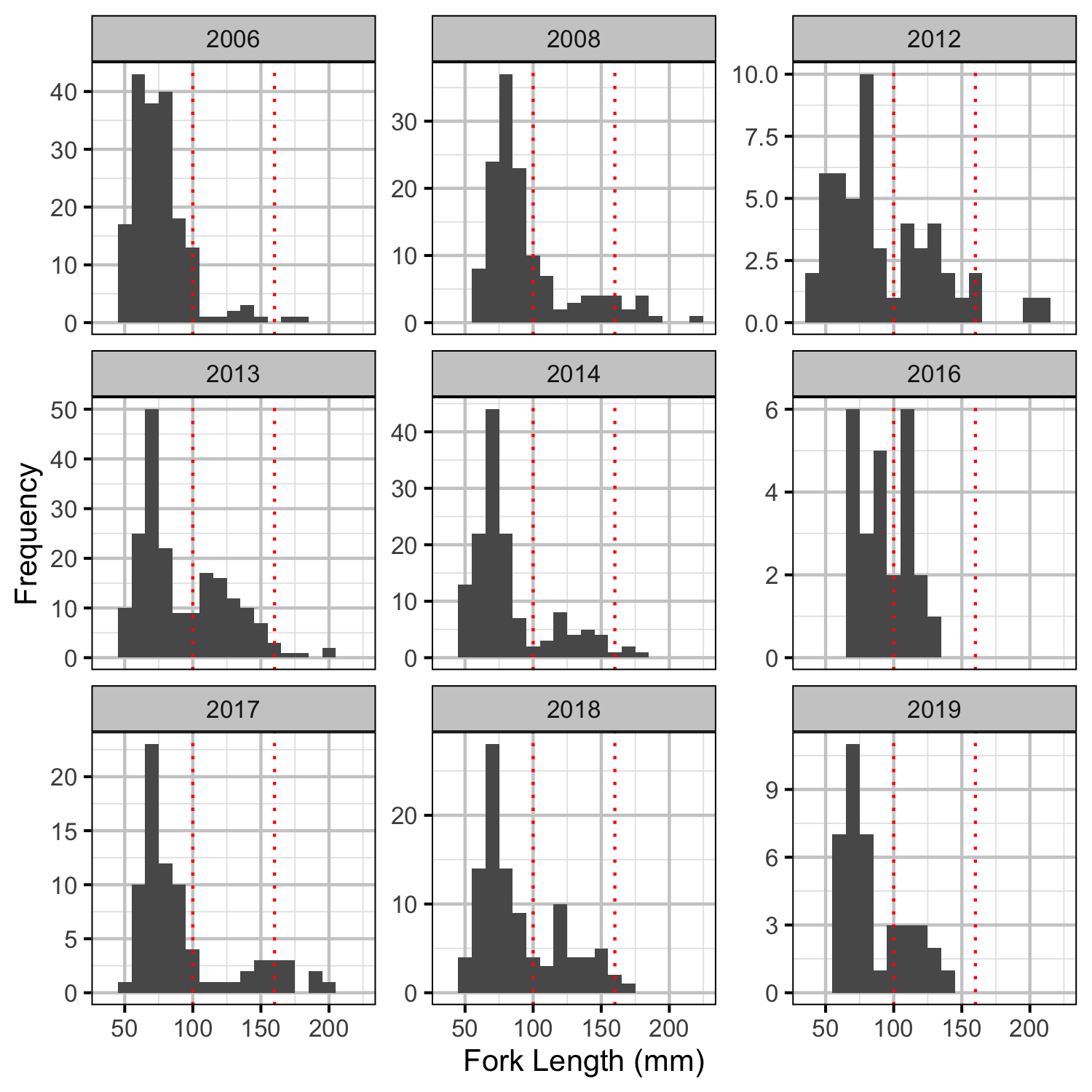Figure 1. Measured length-frequency histogram by year.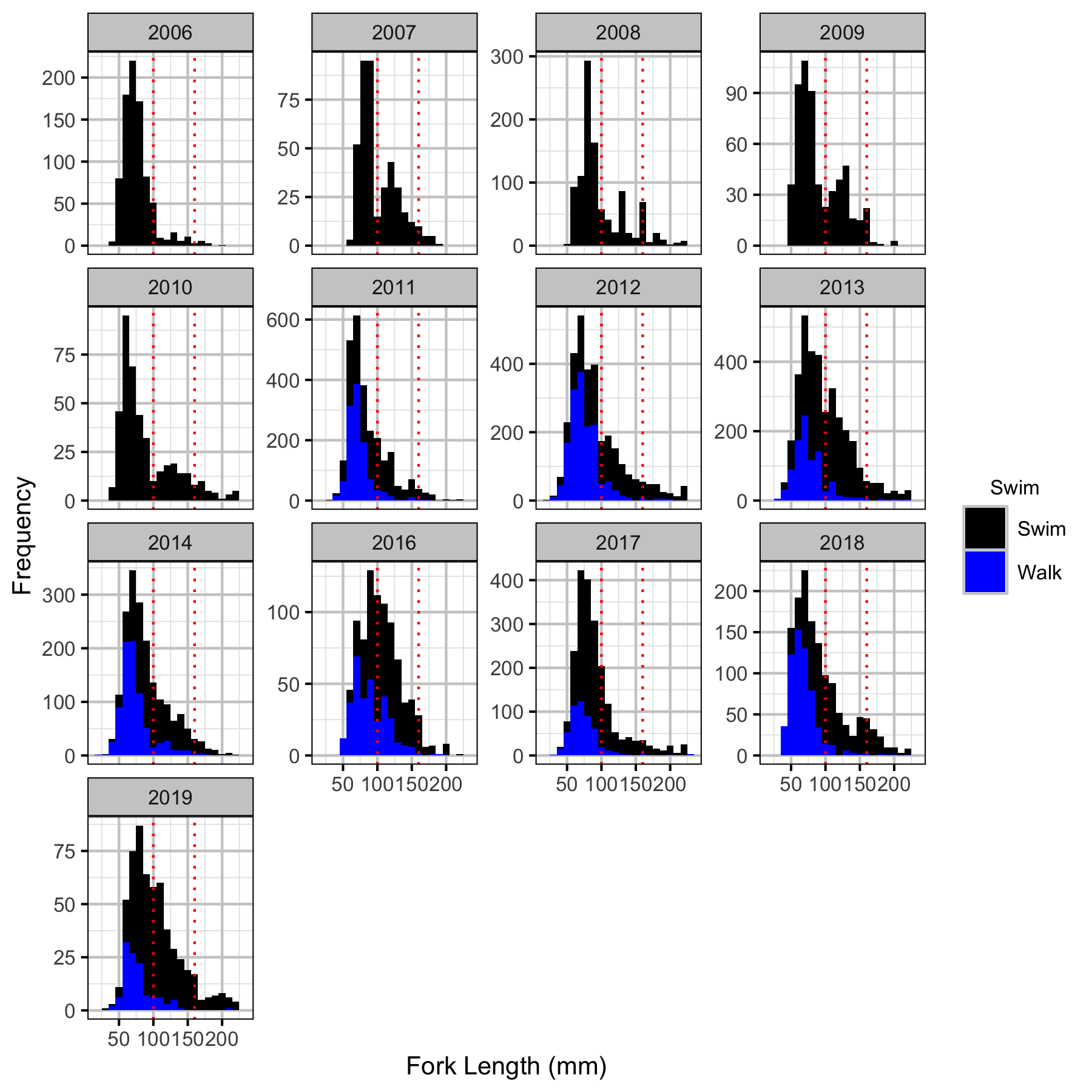Figure 2. Corrected length-frequency histogram by year and observation type.

#### Abundance

##### Age-1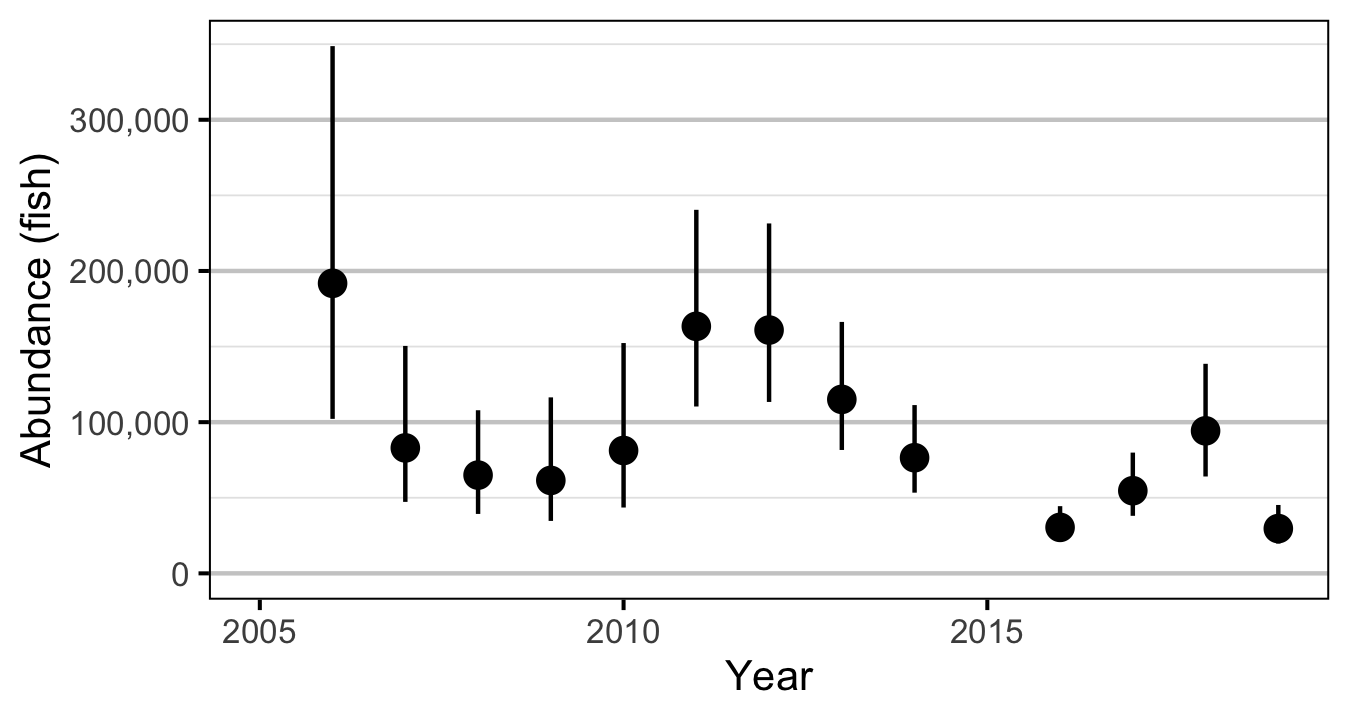Figure 3. Predicted abundance of age-1 Rainbow Trout in the Duncan and Lardeau Rivers by year (with 95% CRIs).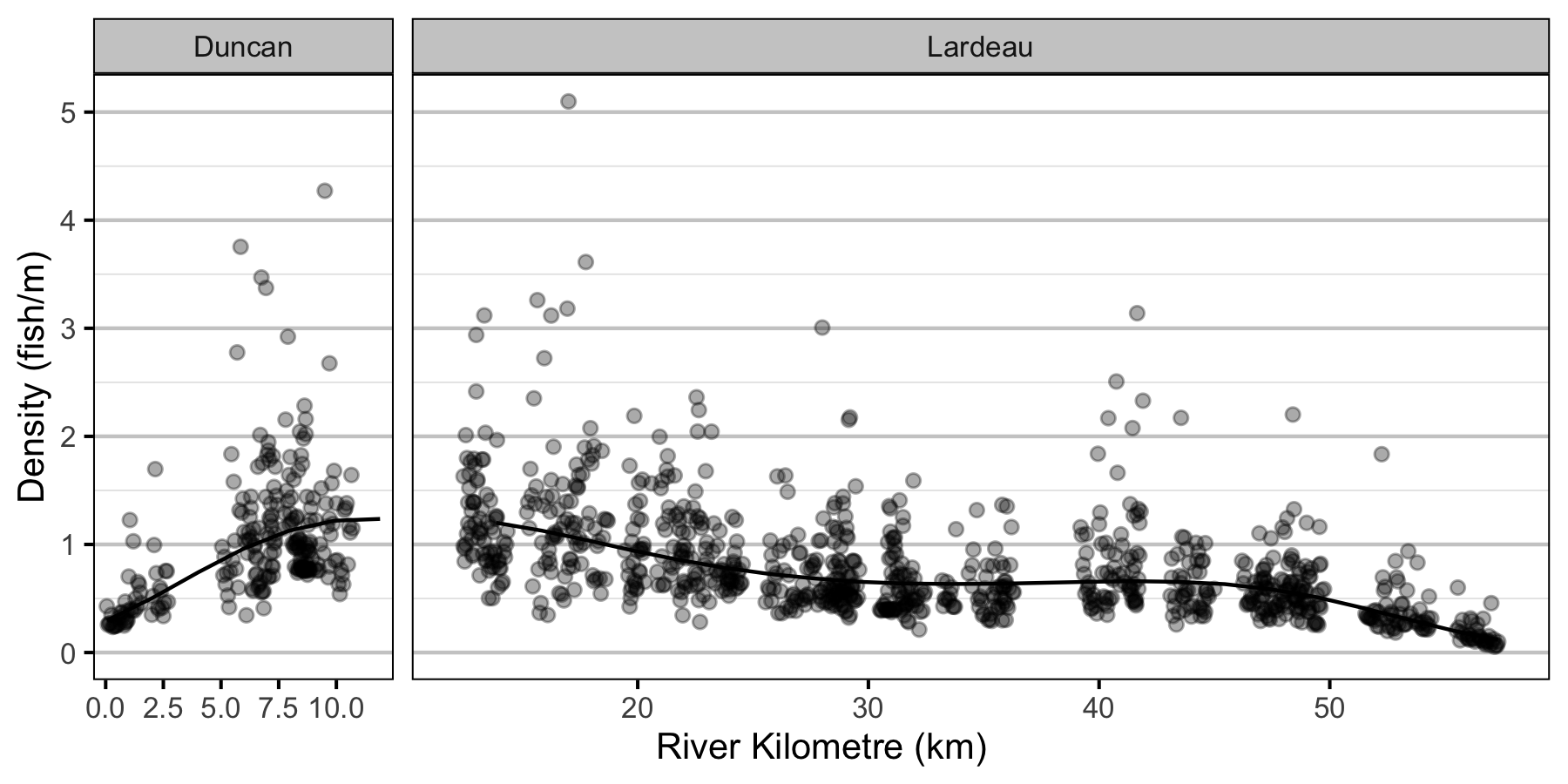Figure 4. Predicted lineal density of age-1 Rainbow Trout in 2010 by river kilometre (with 95% CRIs).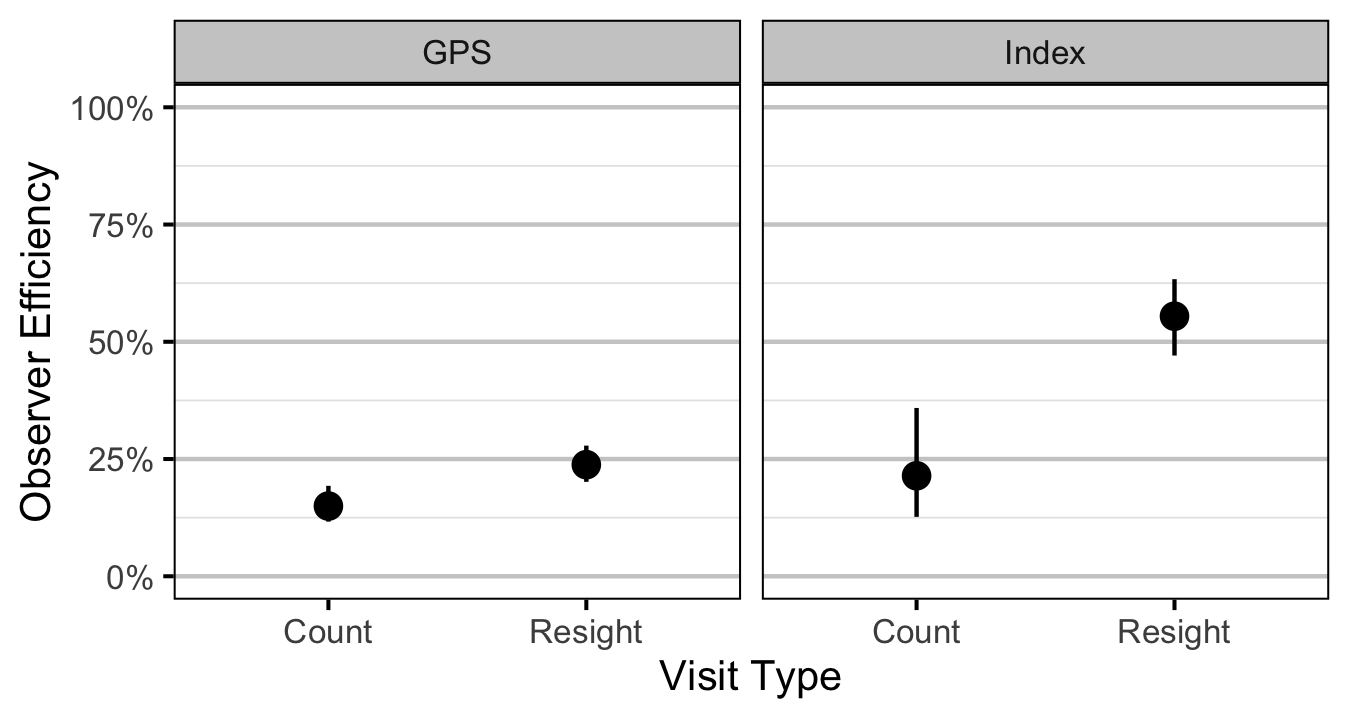Figure 5. Predicted observer efficiency for age-1 Rainbow Trout by visit type and study design (with 95% CRIs).
##### Age-2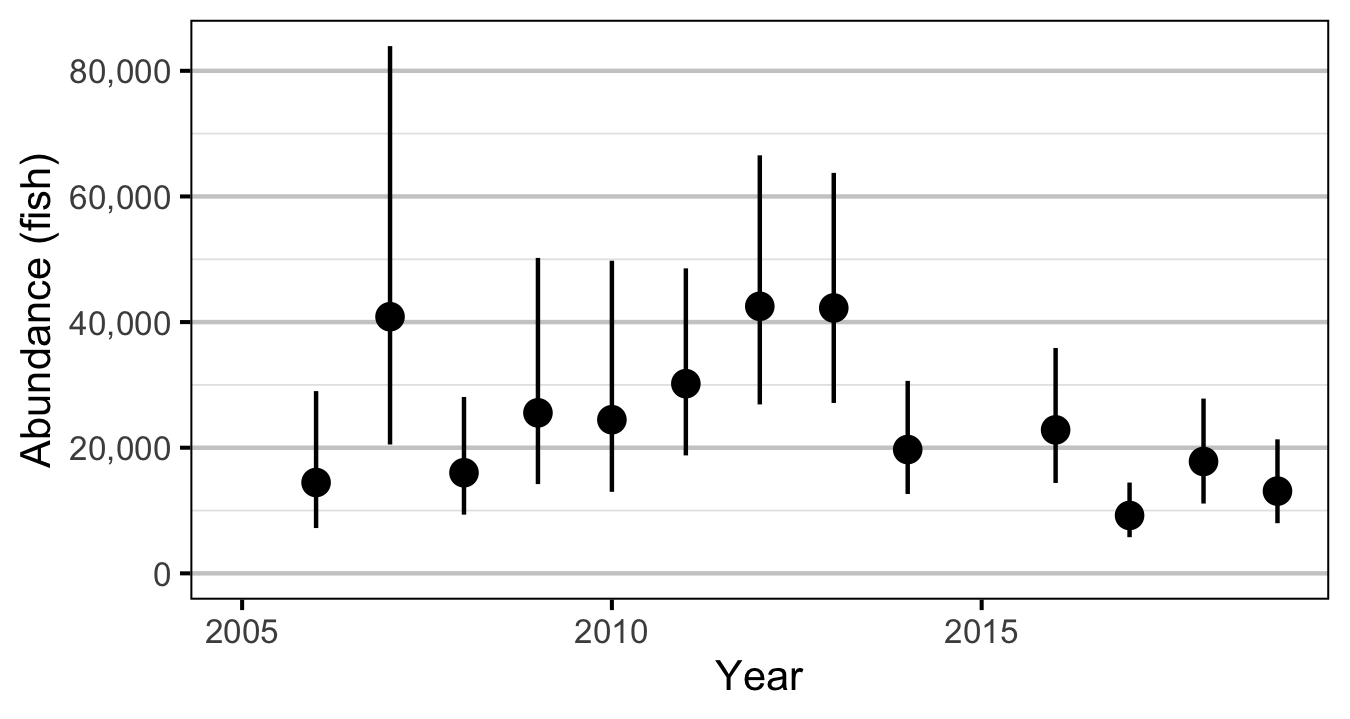Figure 6. Predicted abundance of age-2 Rainbow Trout in the Duncan and Lardeau Rivers by year (with 95% CRIs).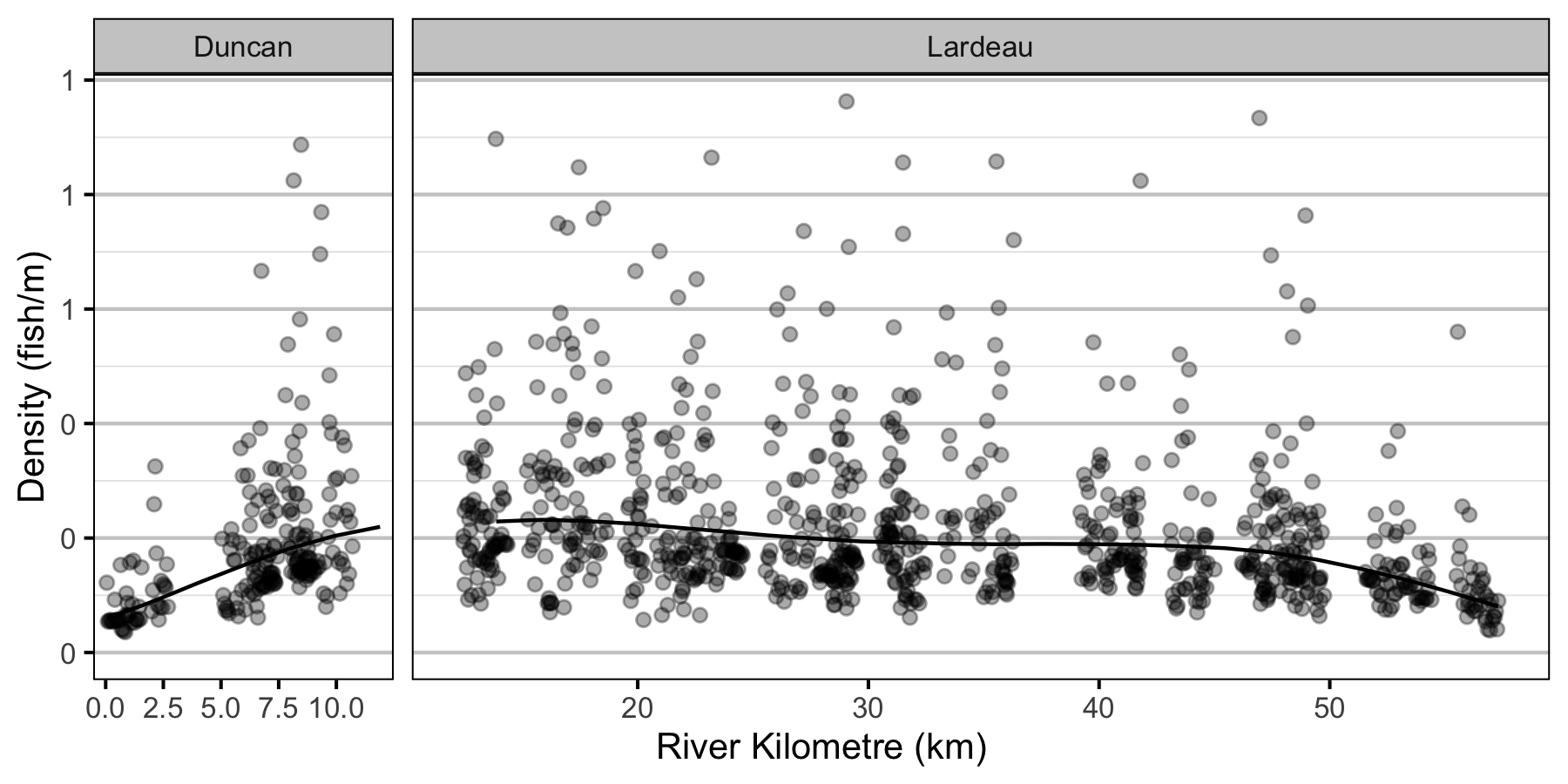Figure 7. Predicted lineal density of age-2 Rainbow Trout in 2010 by river kilometre (with 95% CRIs).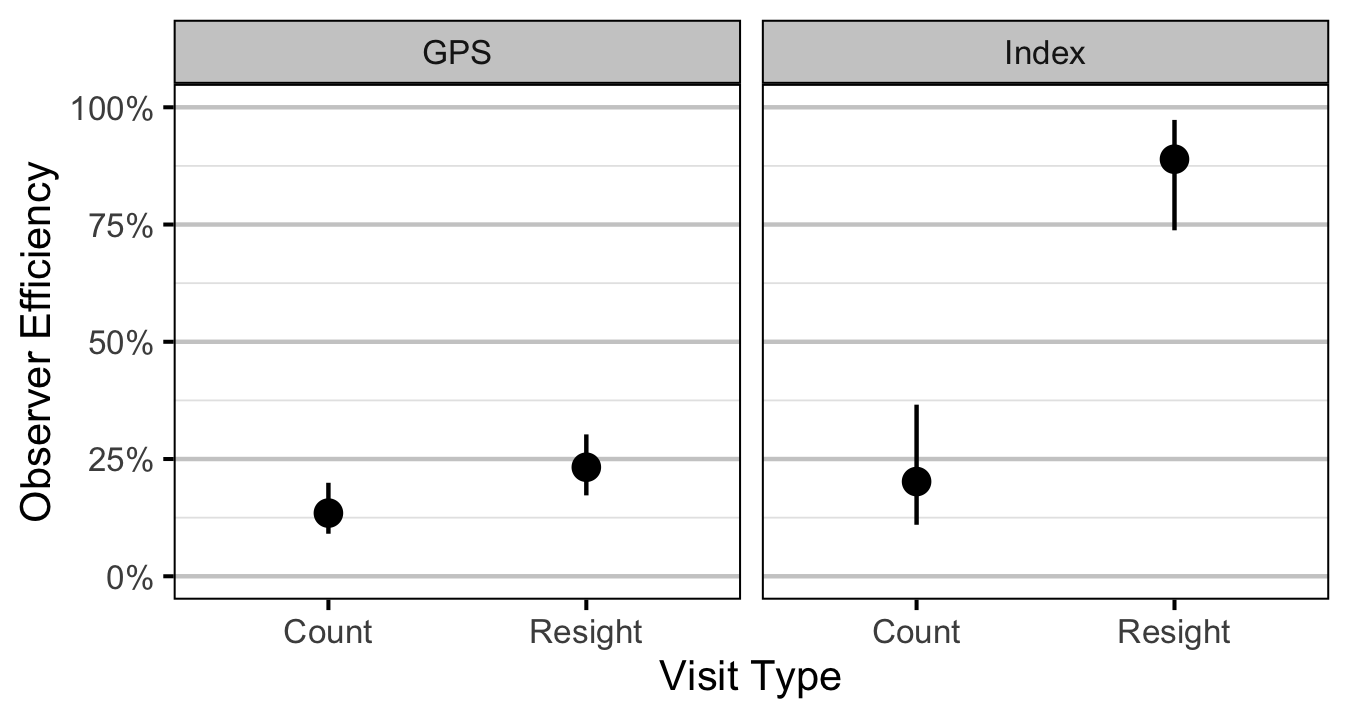Figure 8. Predicted observer efficiency for age-2 Rainbow Trout by visit type and study design (with 95% CRIs).

#### Condition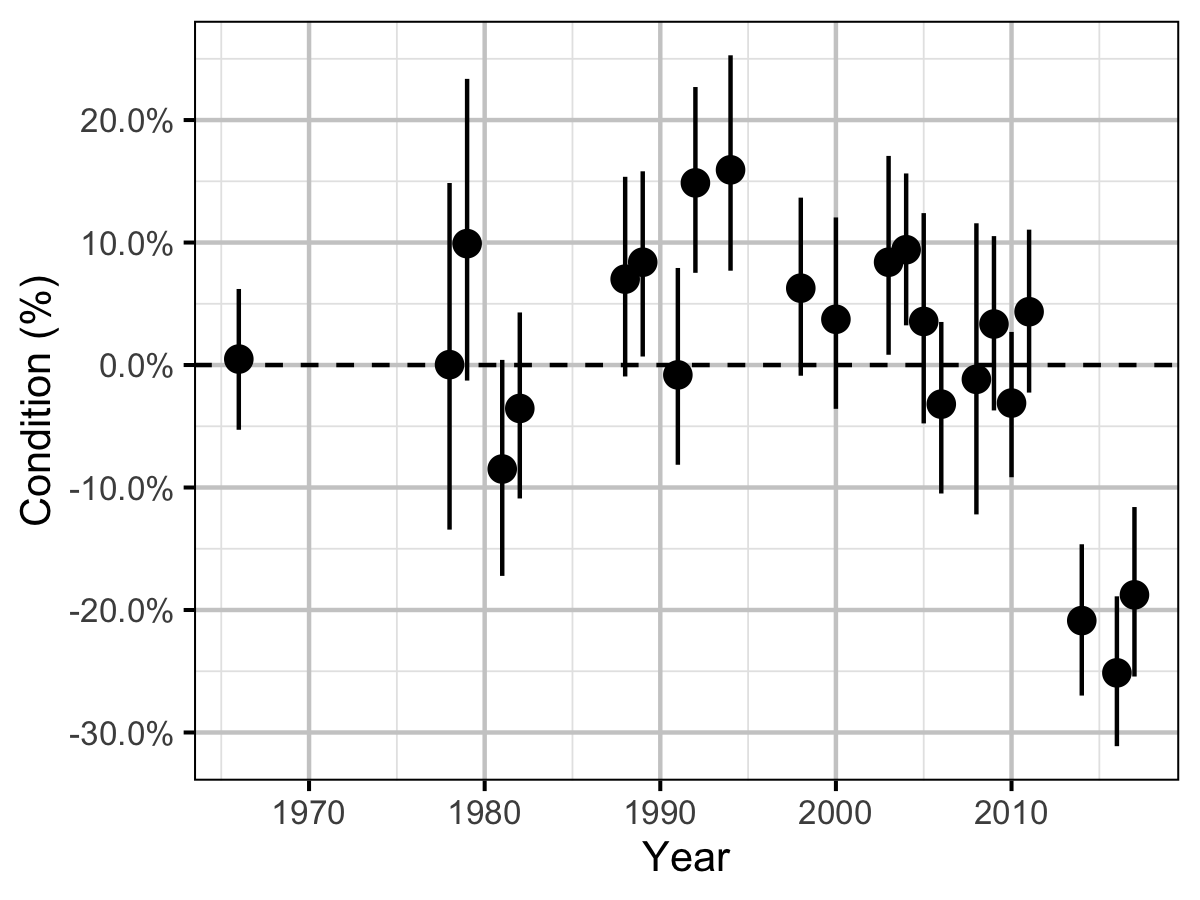Figure 9. The percent change in the body condition for an average length fish relative to a typical year by year (with 95% CRIs).

#### Fecundity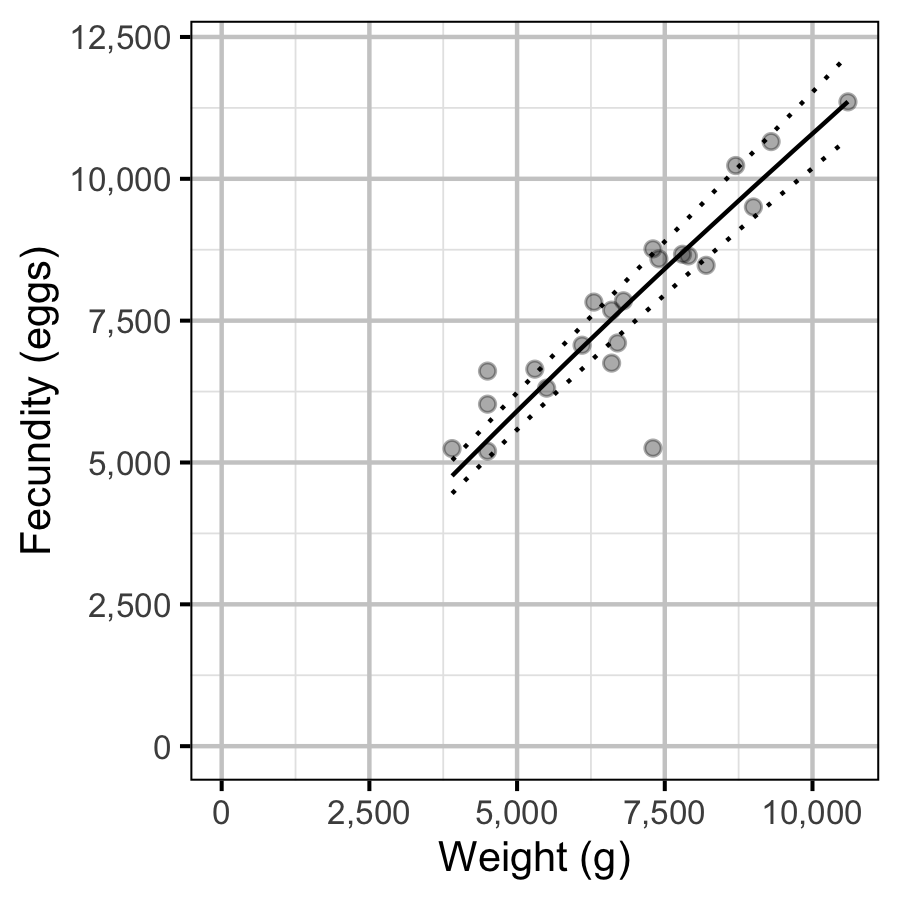Figure 10. The fecundity-weight relationship (with 95% CRIs).

#### Spawner Size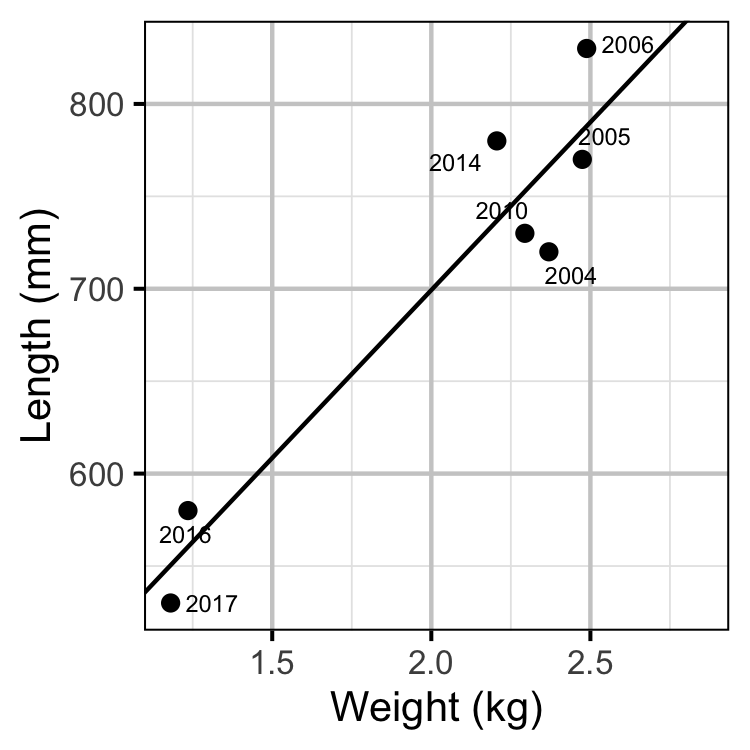Figure 11. The mean length of spawning Rainbow Trout by the mean weight of Rainbow Trout in the KLRT.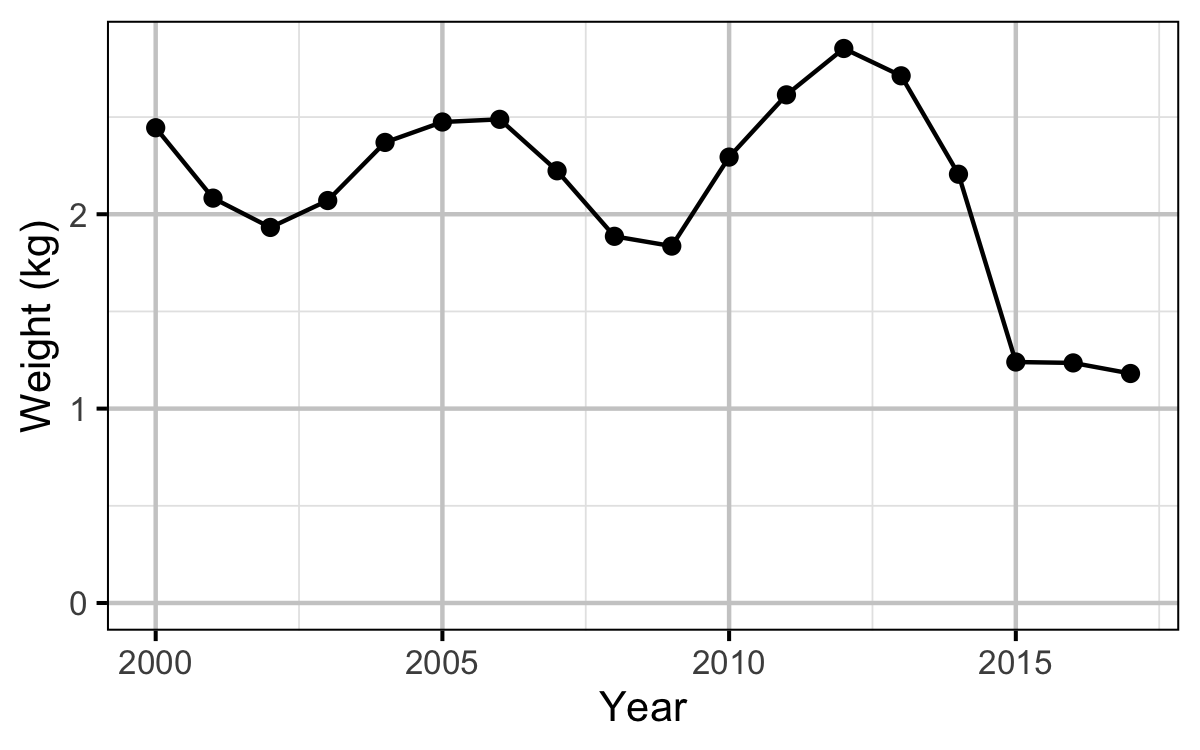Figure 12. The mean weight of Rainbow Trout in the KLRT by year.

#### Egg Deposition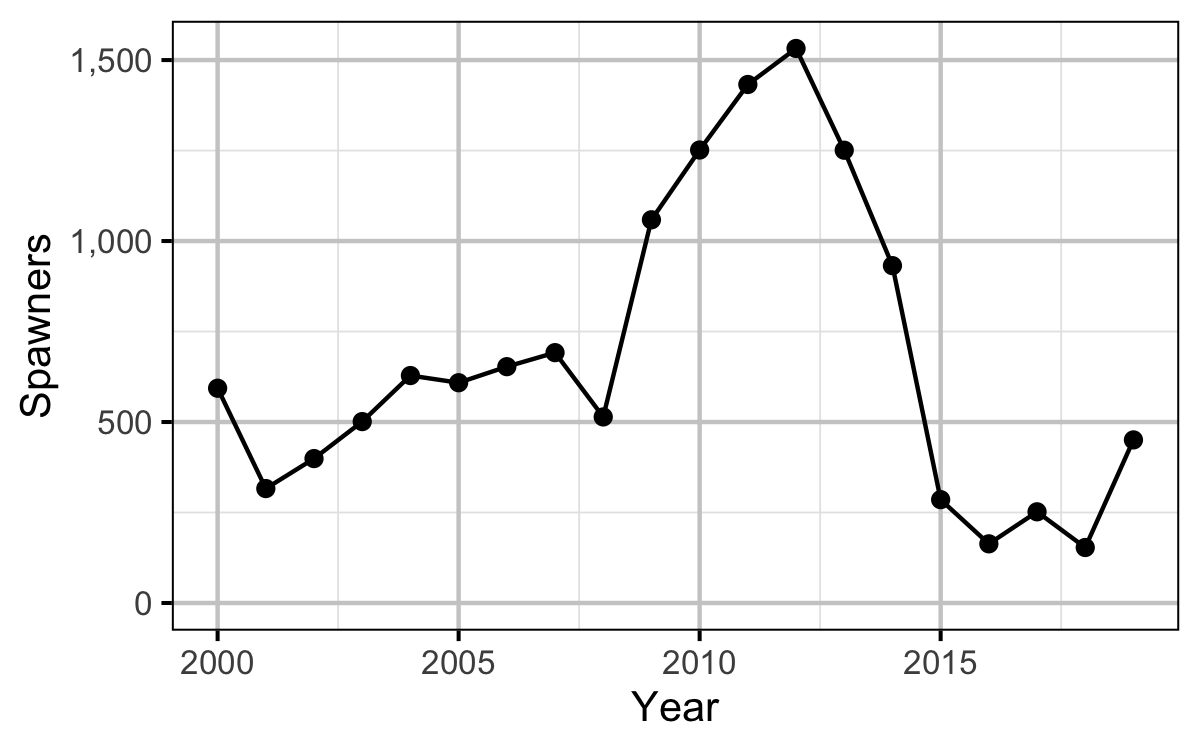Figure 13. The spawner abundance by year.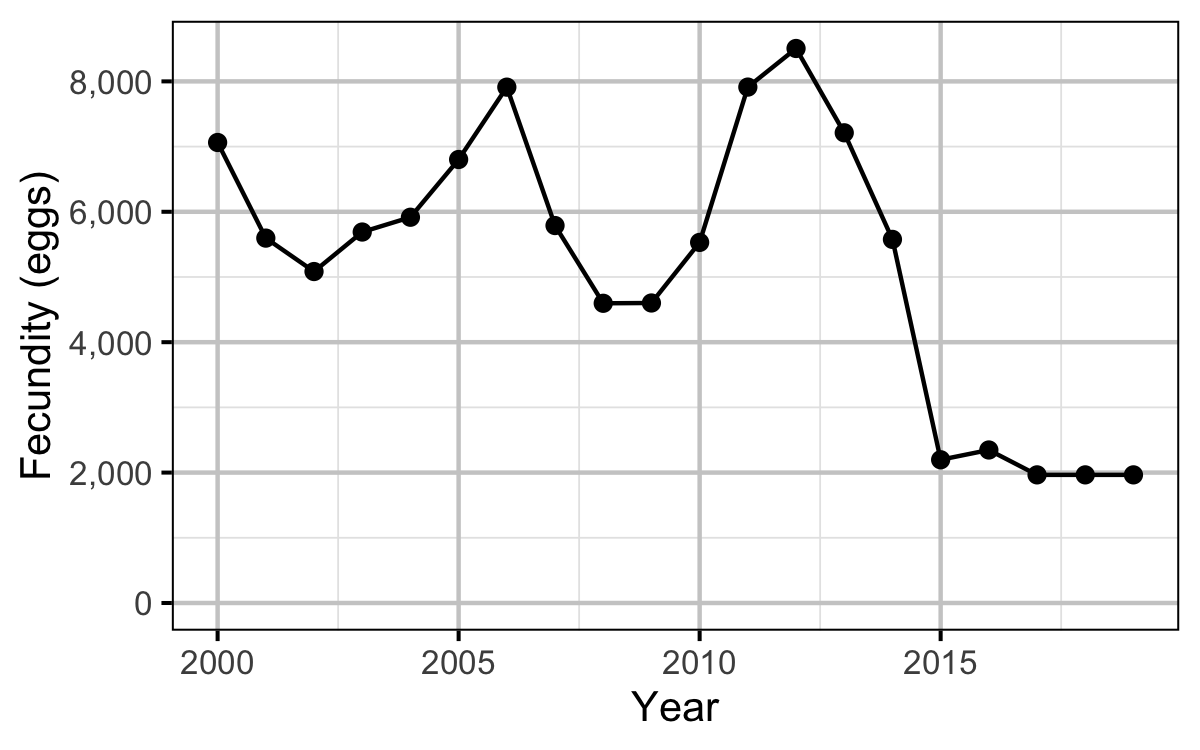Figure 14. The estimated spawner fecundity by year.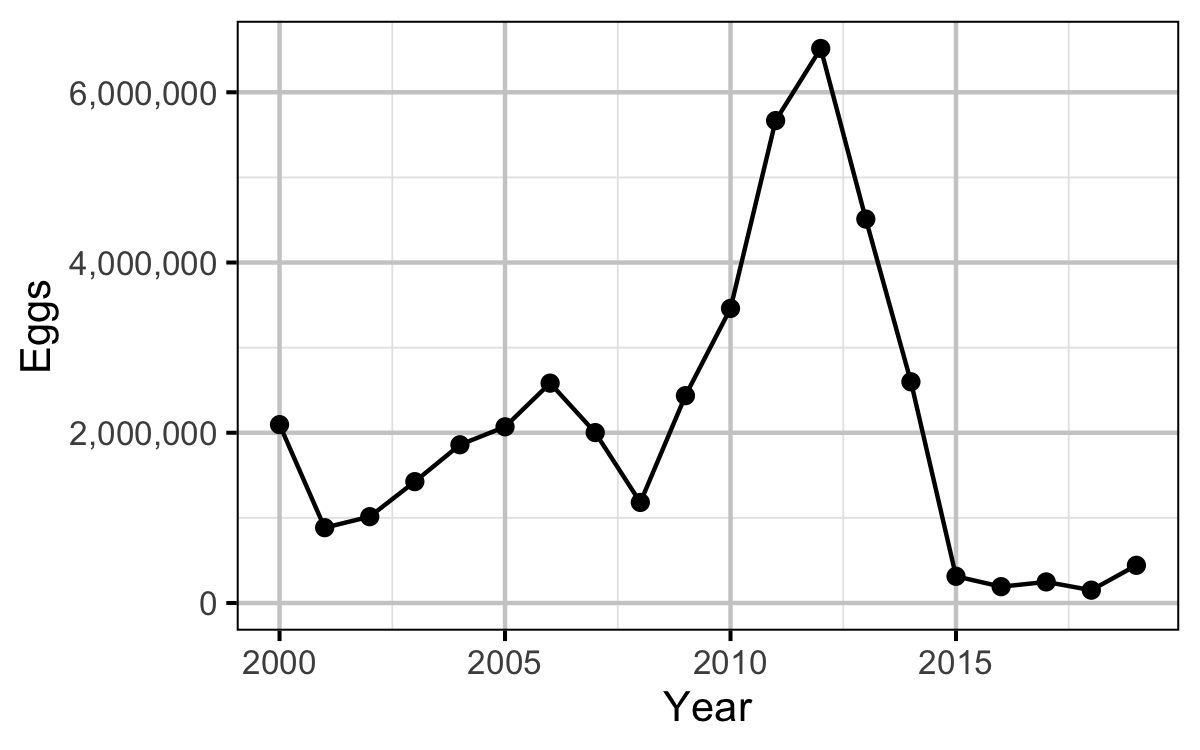Figure 15. The egg deposition by year.

#### Stock-Recruitment

##### Age-1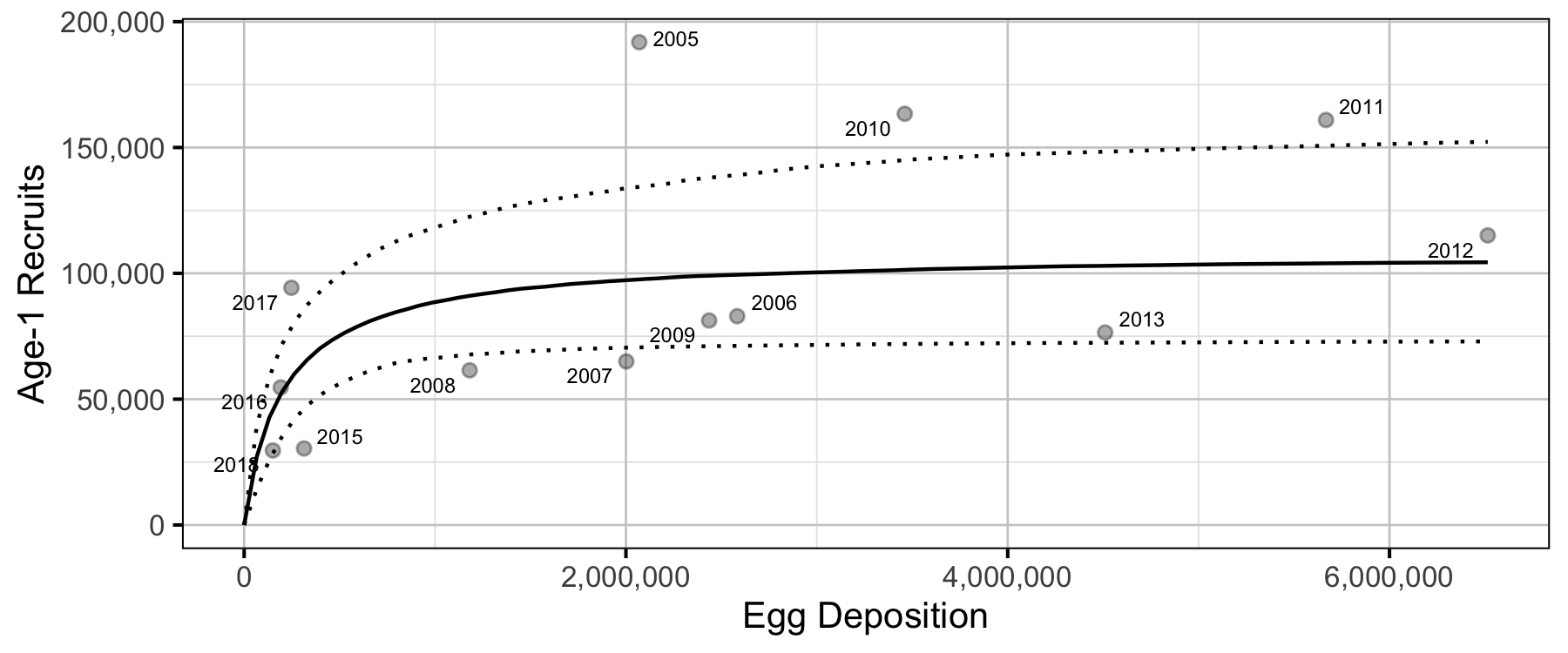Figure 16. Predicted stock-recruitment relationship between spawners and age-1 recruits (with 95% CRIs).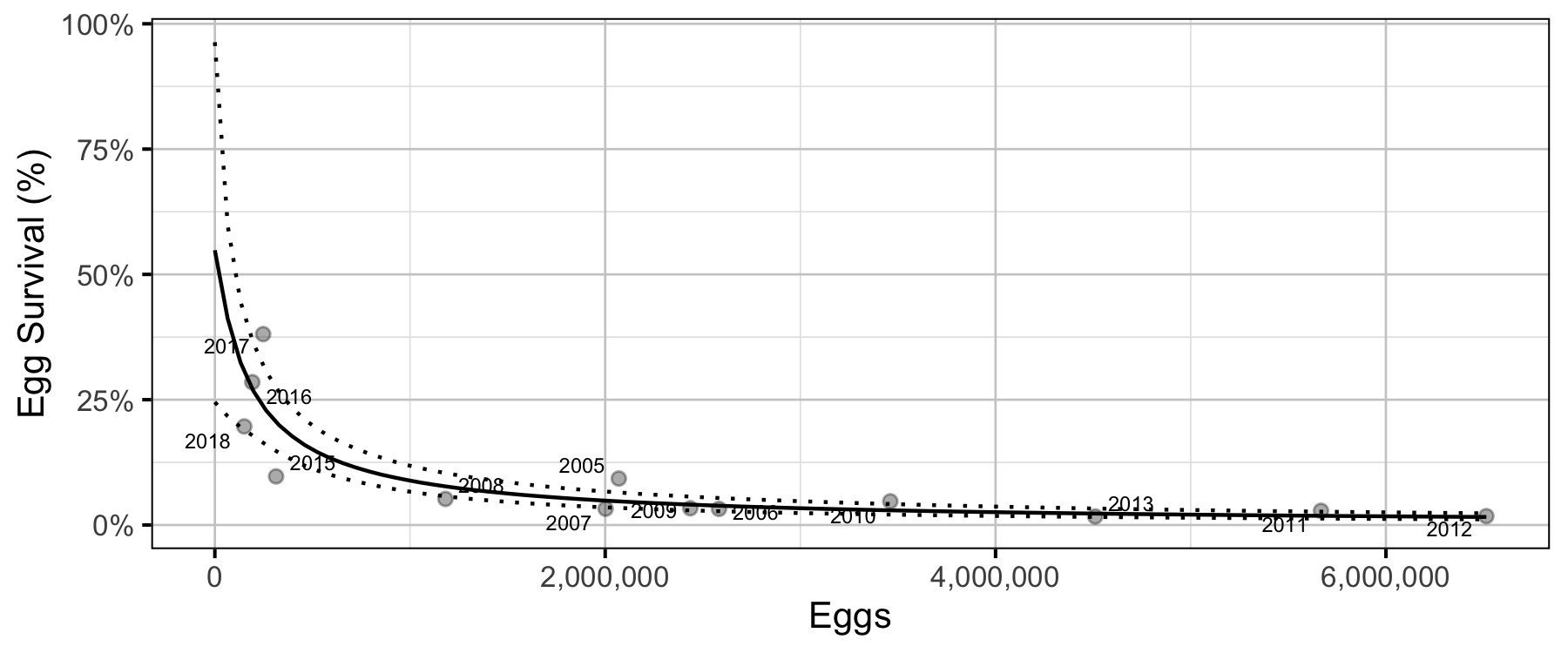Figure 17. Predicted egg survival to age-1 by egg deposition (with 95% CRIs).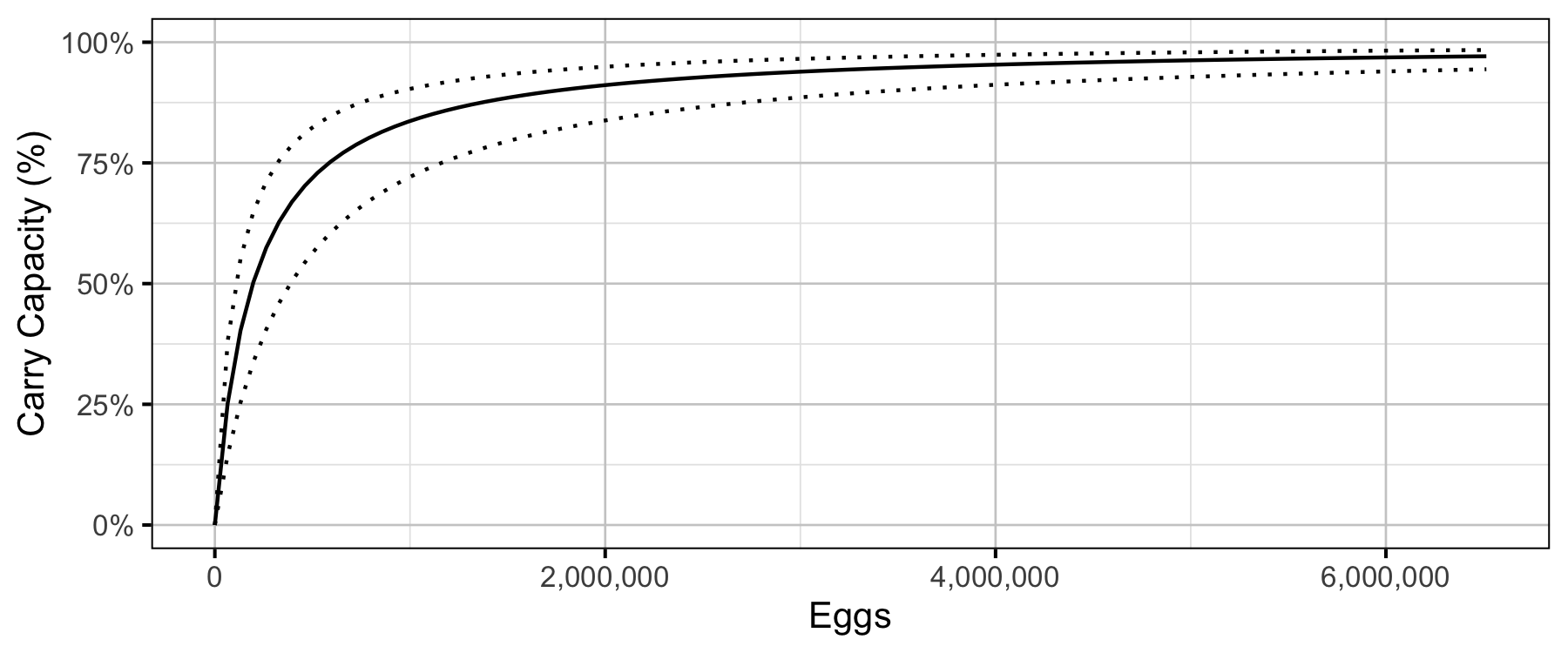Figure 18. Predicted percent of age-1 recruits carry capacity by egg deposition (with 80% CRIs).

#### Age-1 to Age-2 Survival

##### Age-2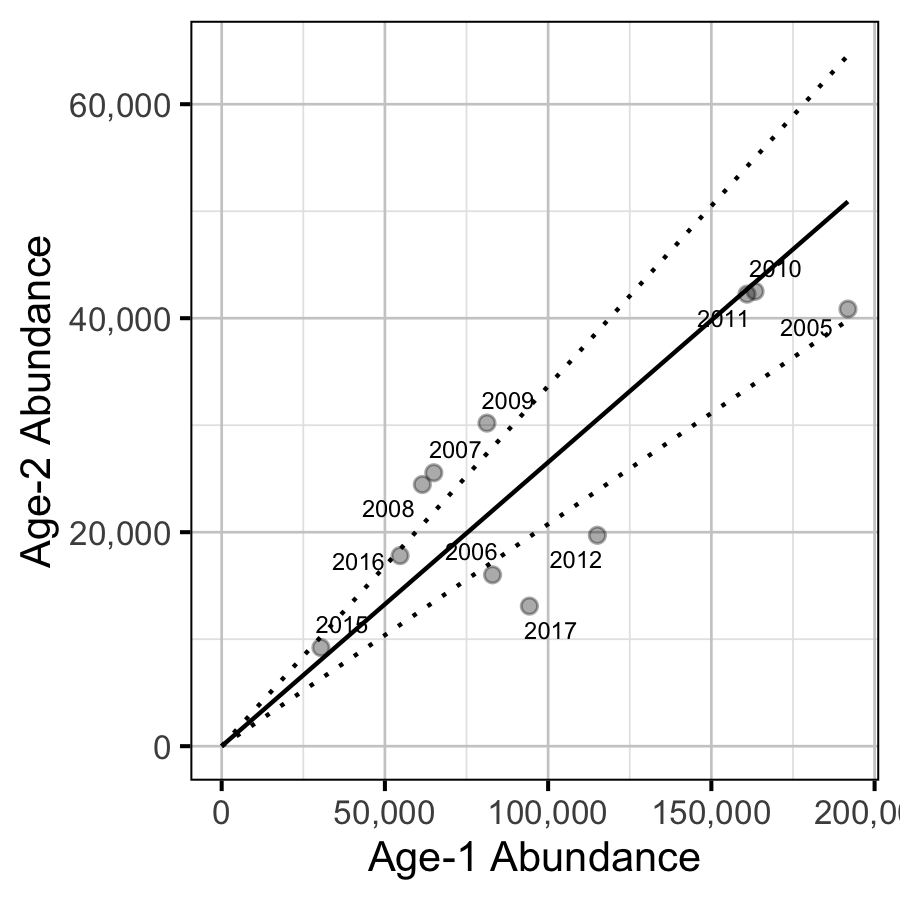Figure 19. Predicted relationship between age-1 and age-2 abundance (with 95% CRIs).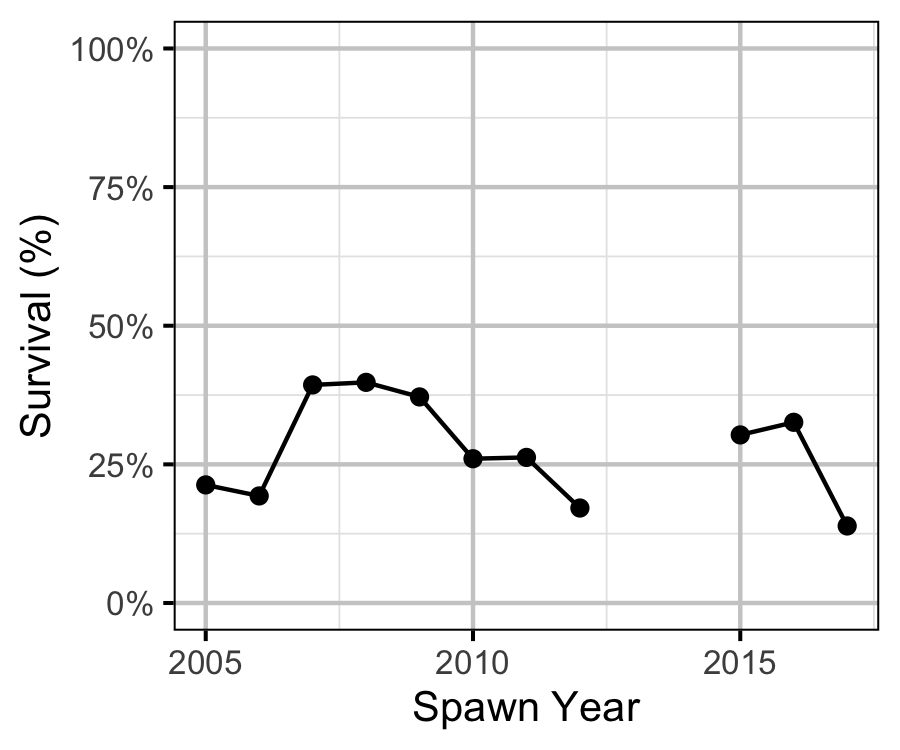Figure 20. Estimated age-1 and age-2 survival by spawn year.

#### Inlake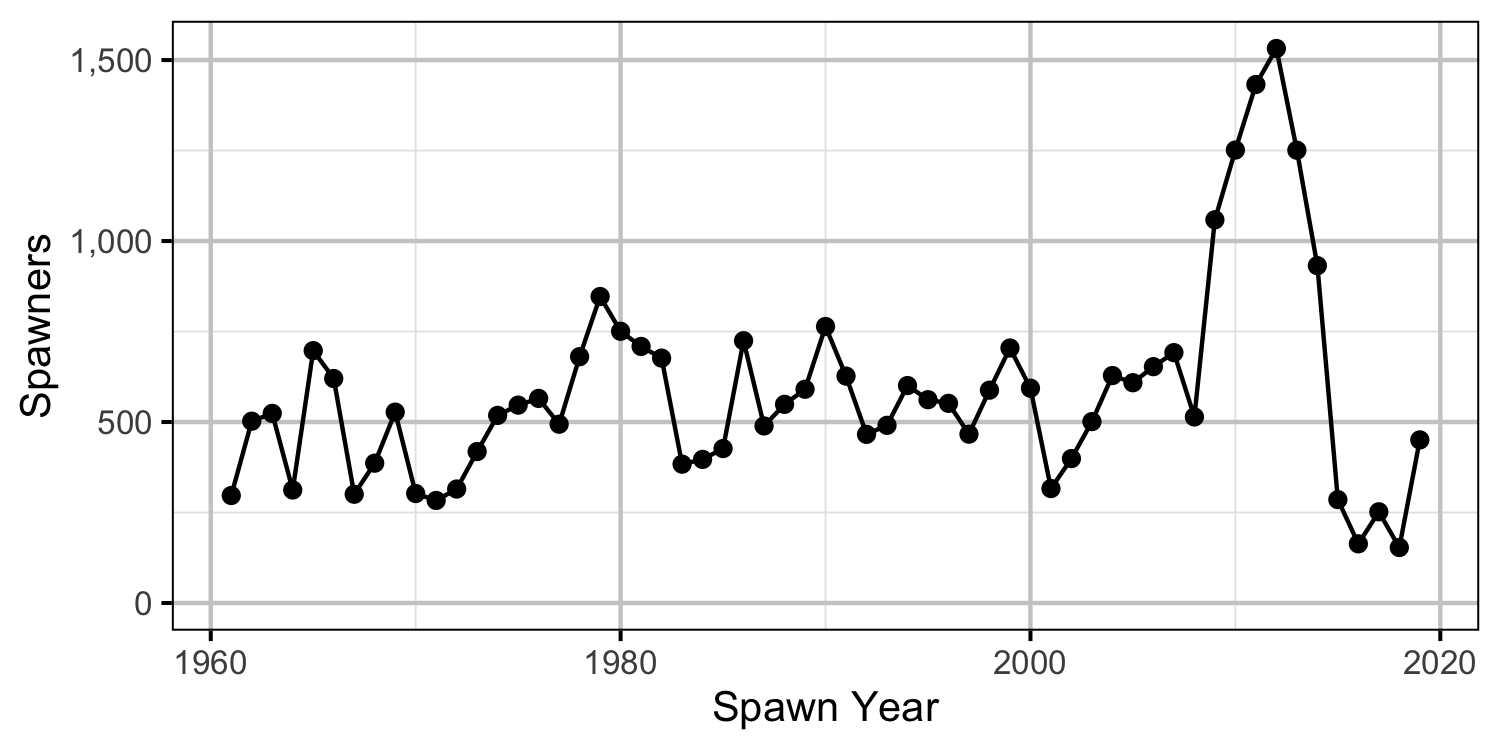Figure 21. The number of spawners by return year.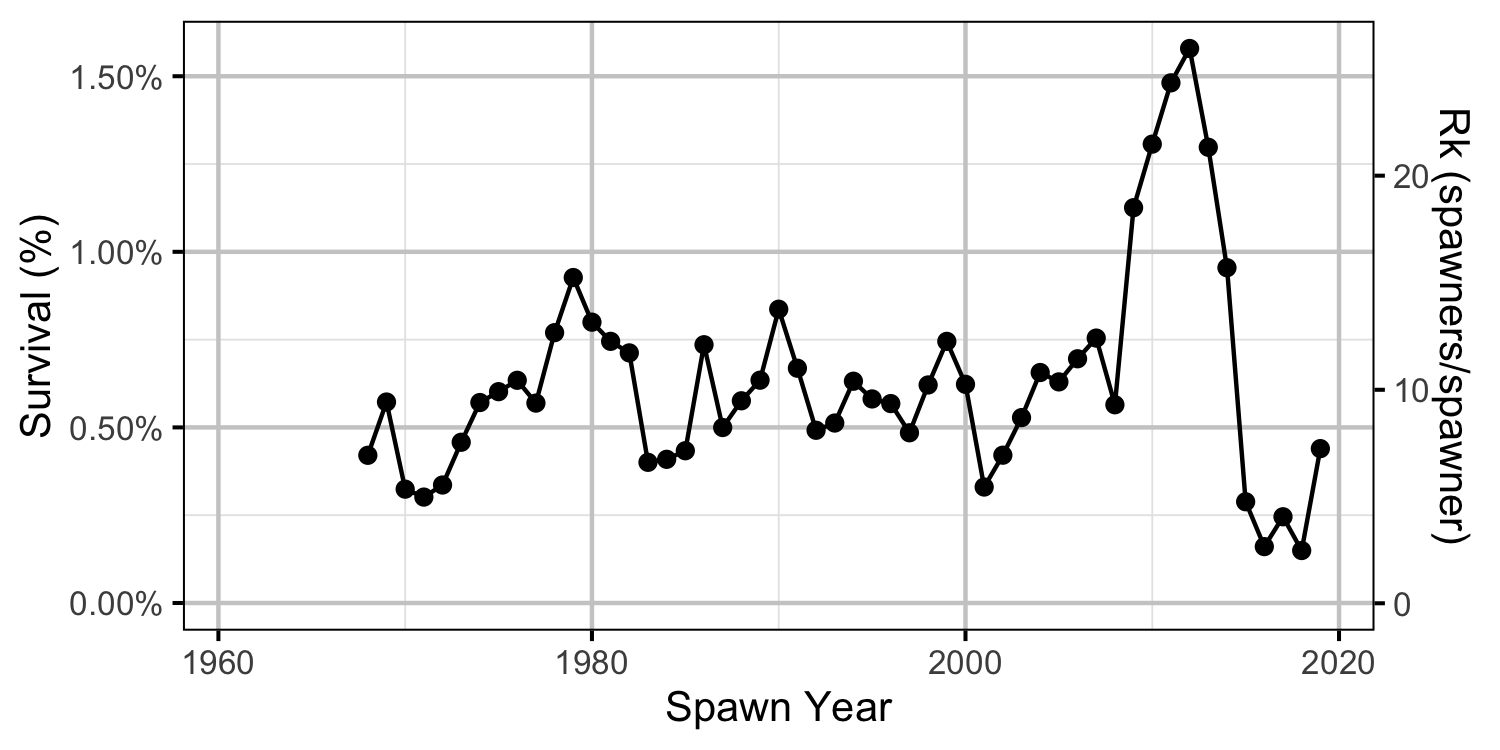Figure 22. Survival from expected age-1 to spawners by return year assuming a fecundity of 6,000 eggs.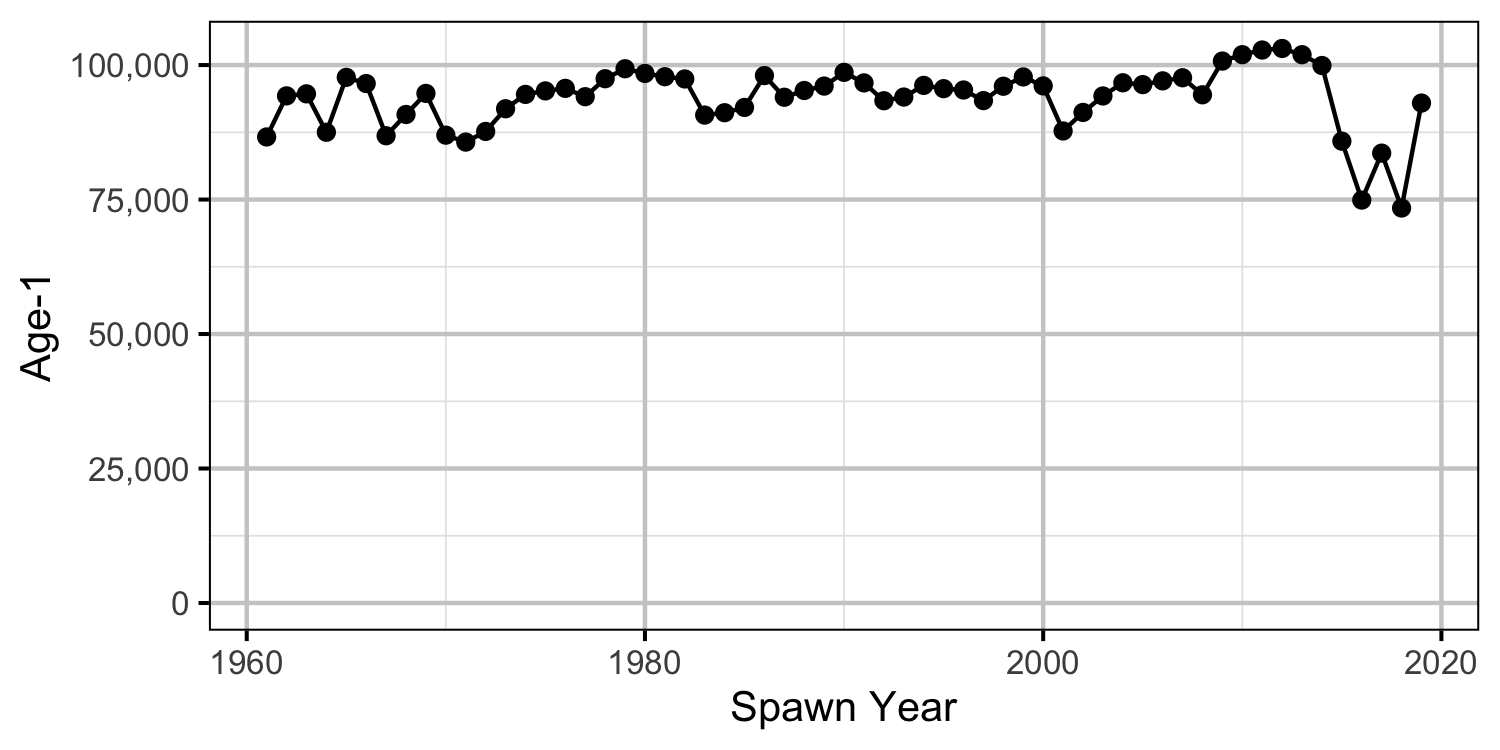Figure 23. The number of expected age-1 recruits by return year assuming a fecundity of 6,000 eggs.

#### Reproductive Rate (age-1)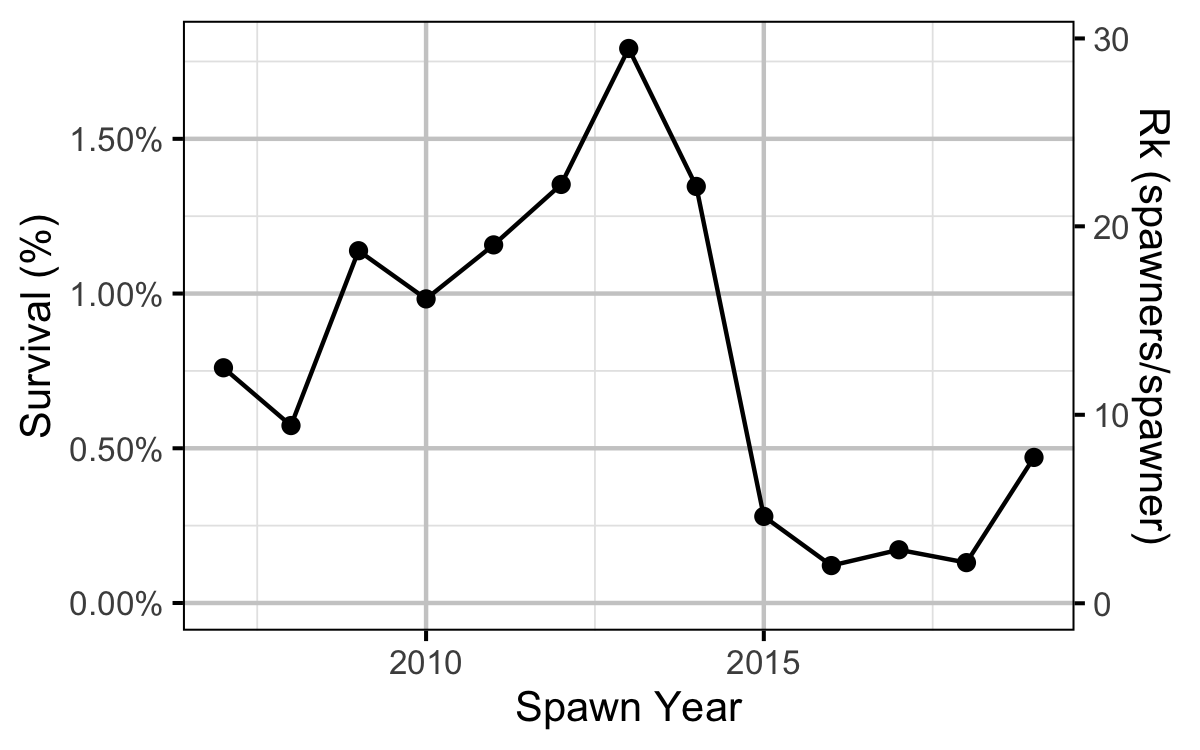Figure 24. Survival from age-1 to spawning by return year.

#### Reproductive Rate (age-2)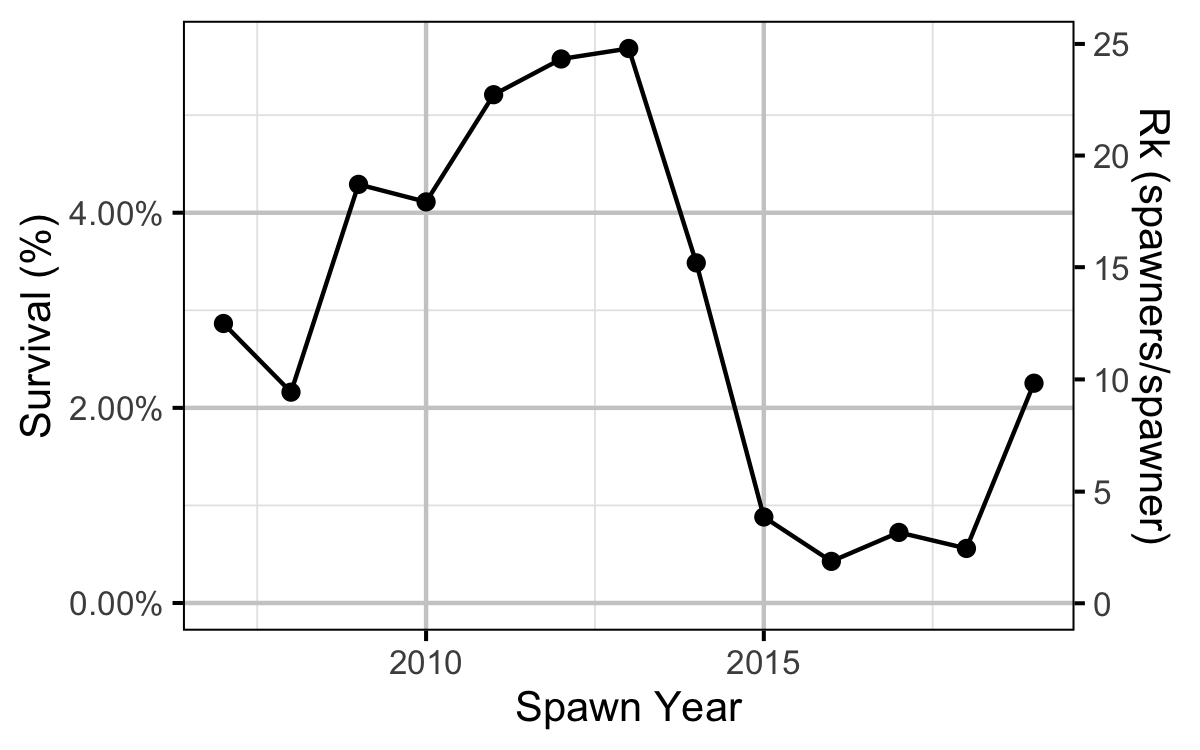Figure 25. Survival from age-2 to spawning by return year.

#### Expected Spawners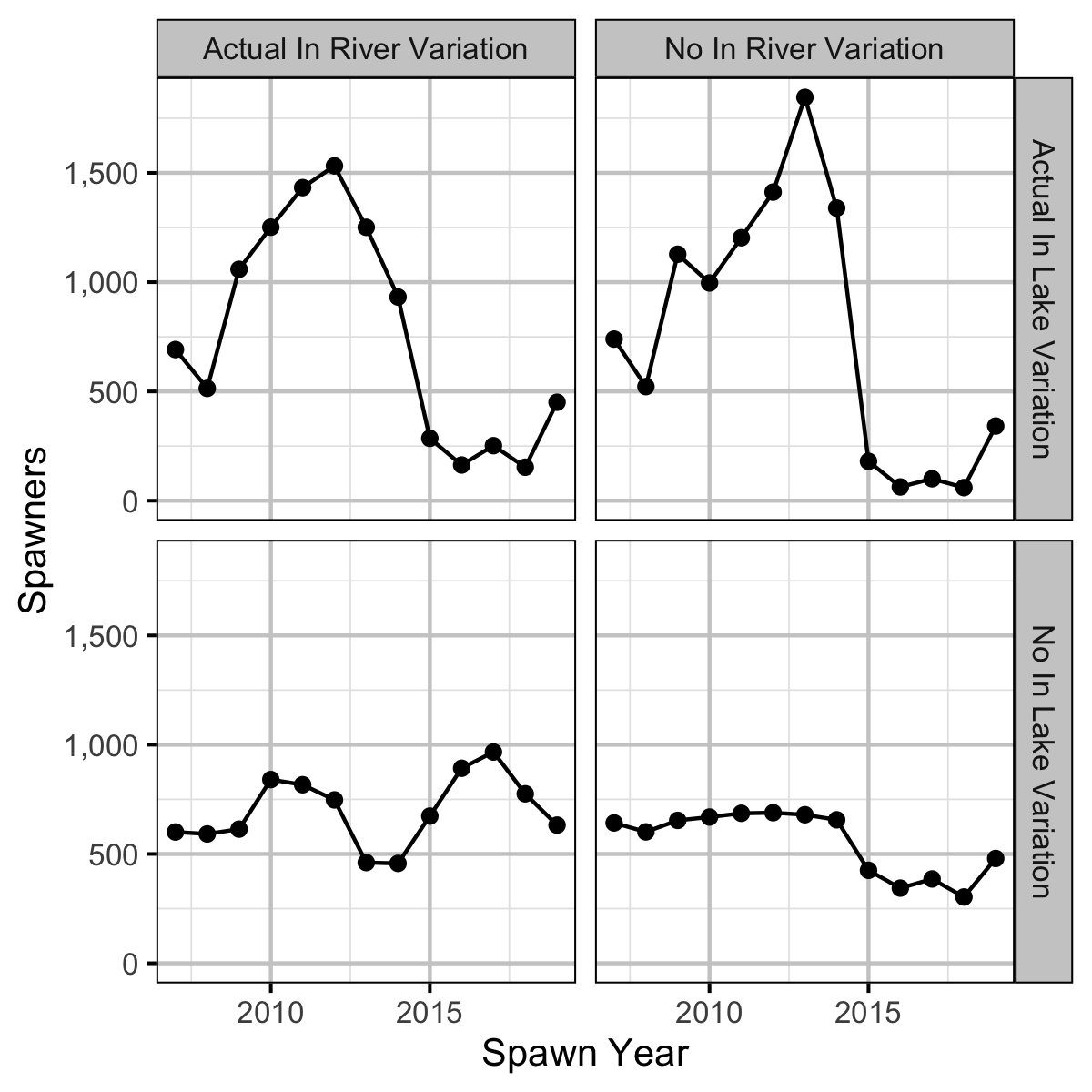Figure 26. Expected spawners by return year and in river and in lake variation based on age-1 recruitment.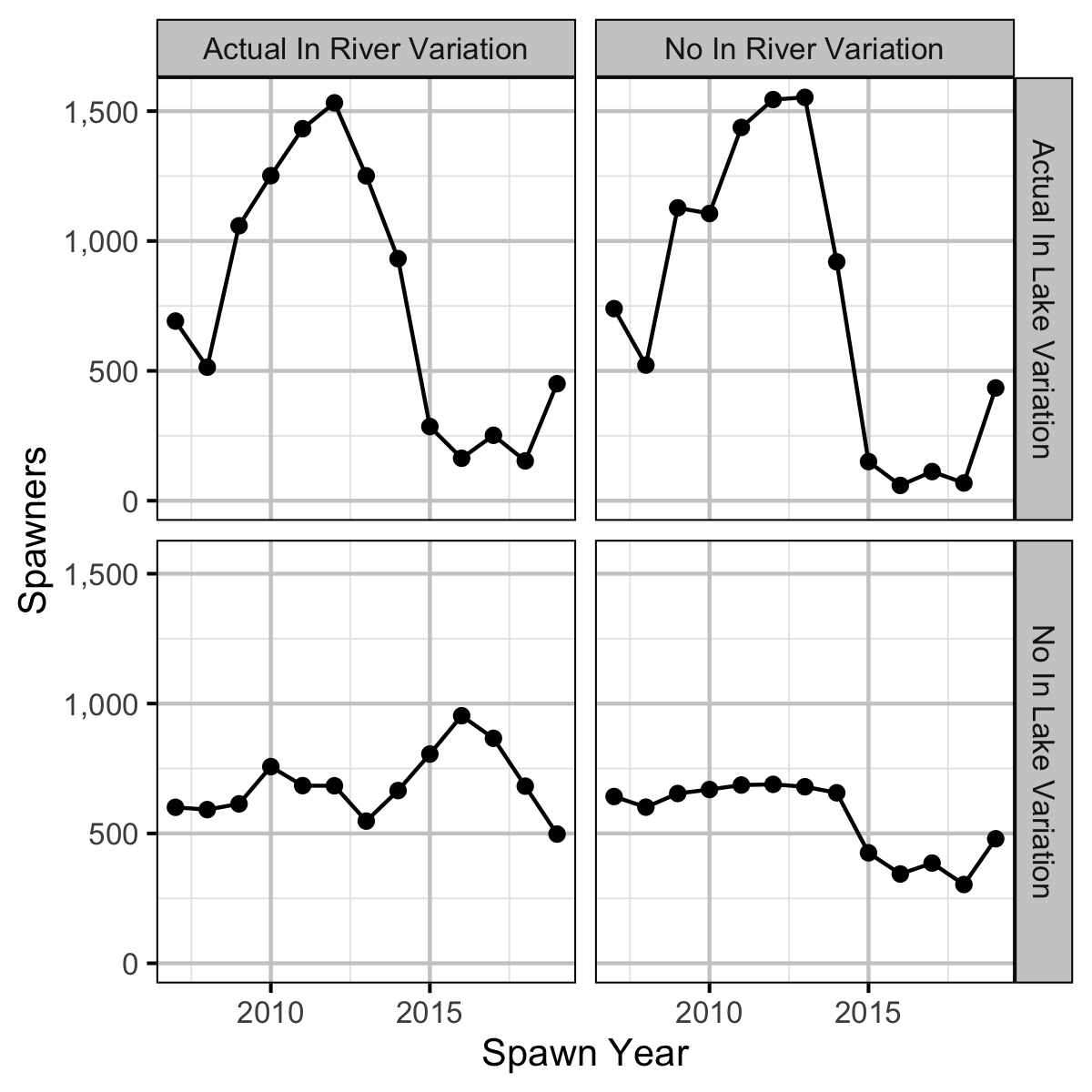Figure 27. Expected spawners by return year and in river and in lake variation based on age-2 recruitment.

#### Conclusions

• Modeling the density in each year as a random effect (as opposed to a fixed effect) substantially reduced the estimated density for 2006.
• The apparent survival from age-1 to age-2 was a density-independent 27%.
• The Limit Reference Point is 387,000 eggs or 129 AUC spawners (based on fecundity in a typical year).
• The egg deposition has fallen below the LRP since 2015.
• Although the 2005 spawn year resulted in double the expected recruitment, the following four years were all below average.
• Calculations suggest that the spike in the number of spawners was solely due to changes in the inlake survival.

### Recommendations

• Develop a Limit Reference Point that ensures a effective population size ($$N_e$$) of 500.
• Develop an Upper Reference Point that ensures adequate Kokanee recruitment.

## Acknowledgements

The organisations and individuals whose contributions have made this analysis report possible include: